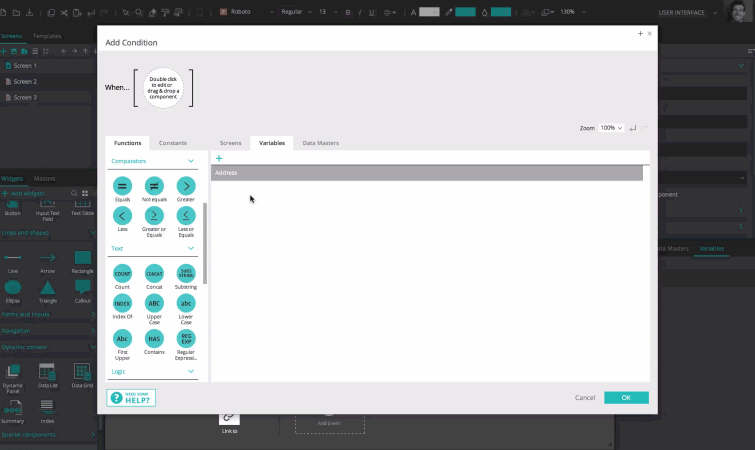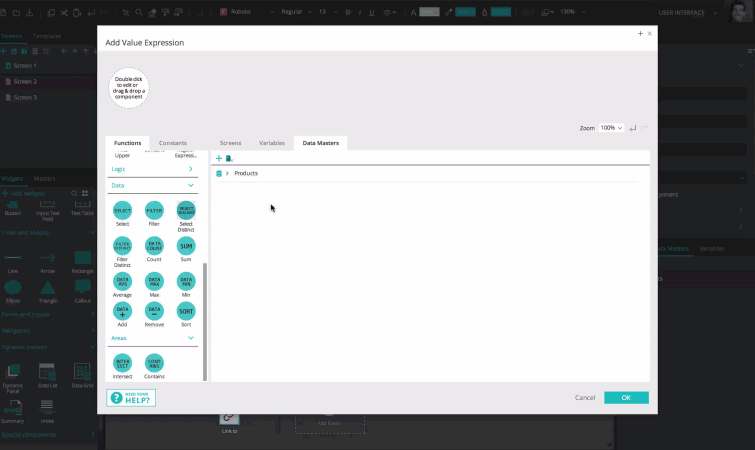# Calculated values and conditions

Both the Value Expression builder and the Conditional Expression builder can be used to create advanced values, conditions or filters.

The Value Expression builder results in a value or list of values, while the Conditional Expression builder results in “True” or “False”.

The Value Expression builder will appear with a blank space in which to start building the value, while the Conditional Expression builder will appear with a “When…”, with the blank space next in which to start building the condition.

The Value Expression builder can be accessed by clicking on the “Calculated” value option for the following actions:

• Set Value
• Rotate
• Resize
• Move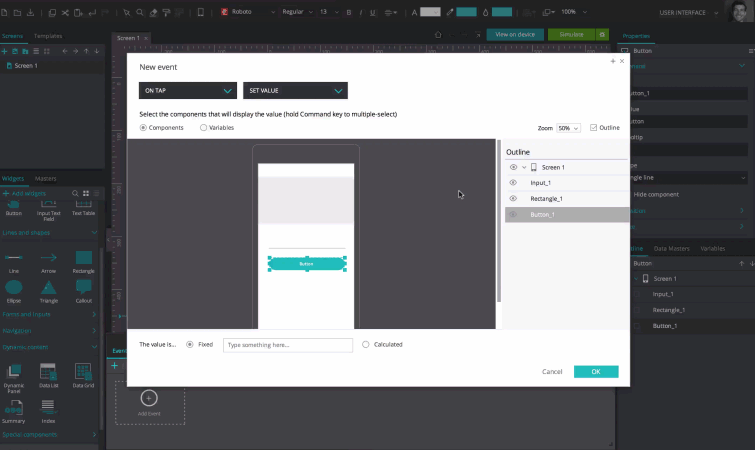The Conditional Expression builder can be accessed by clicking on “Add condition” or “Else add condition” after creating an event.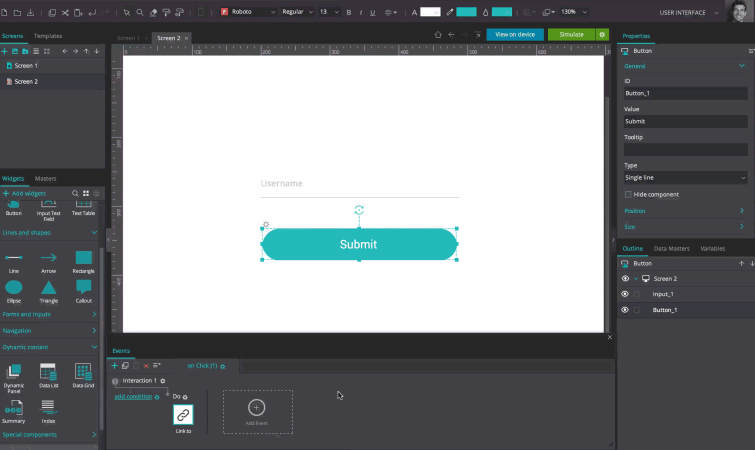## The expression builder broken down

Here’s the builder broken down, using the Conditional Expression builder as the example: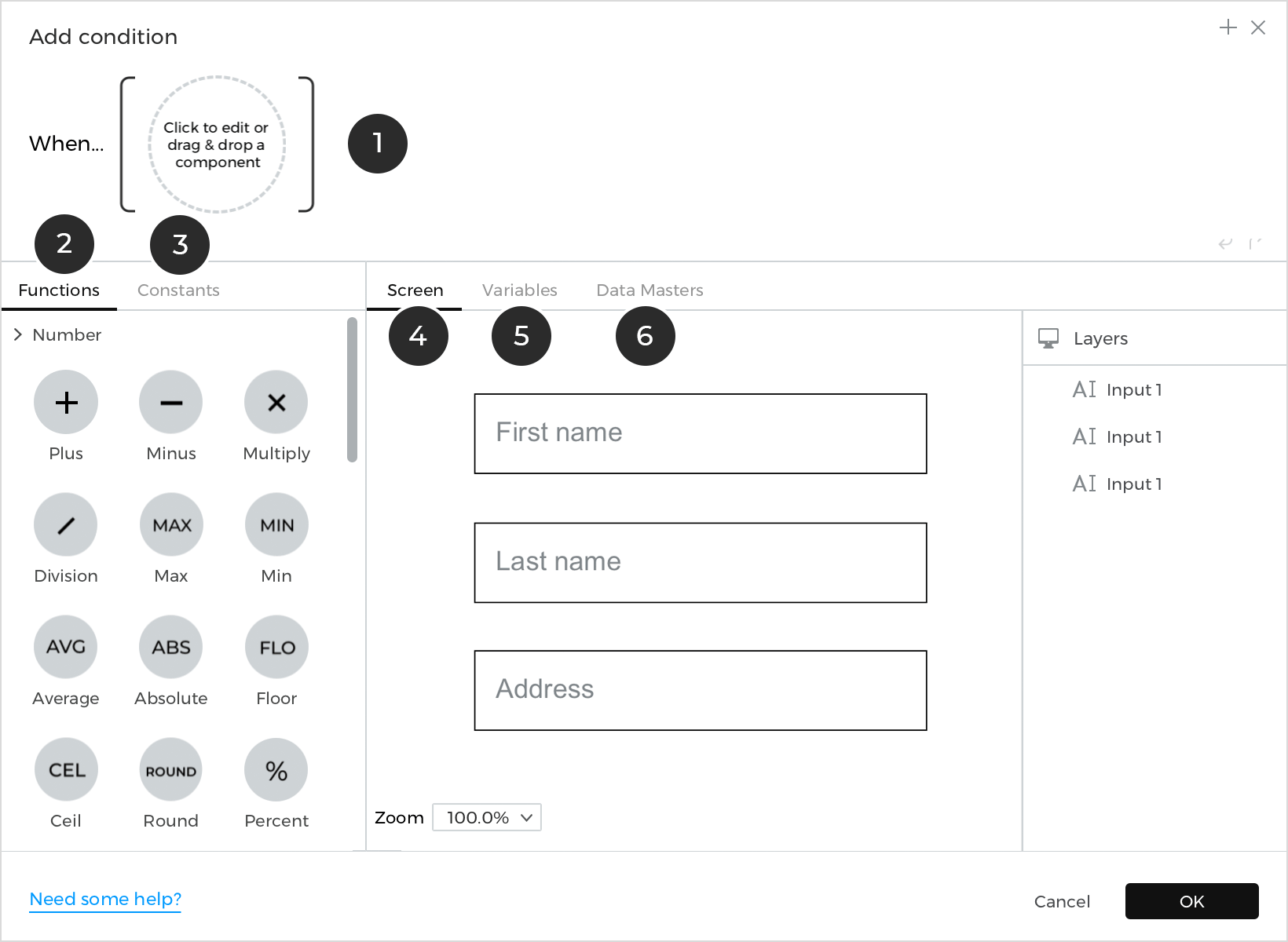1. The expression: this is where conditions are built
2. Functions: these are combined with UI elements, variables, Data Masters (or Data Master fields), text or numbers to build conditions
3. Constants: these are fixed values that can be used on their own (or combined with functions, UI elements, variables, Data Masters, text and numbers) to build a condition
4. Screens: the default tab displayed on the Canvas within the builder. From here, choose which UI element(s) to include in a condition.
Note: only the UI elements in the current screens will be available for selection
5. Variables: select this tab to choose a variable to include in a condition
6. Data Masters: select this tab to choose a Data Master (or Data Master field) to include in a condition

Conditions and calculated values are built by dragging a combination of any of the following to the expression: functions, constants, UI elements, variables, Data Masters (or Data Master fields) and text strings.

The following are the built-in tools to be used in the Value Expression and Conditional Expression builders:

## Functions in the expression builder

Functions are divided into number, comparator, text, logic, data and area functions.

### Numbers

Number functions relate to numbers and the various ways of computing numeric values: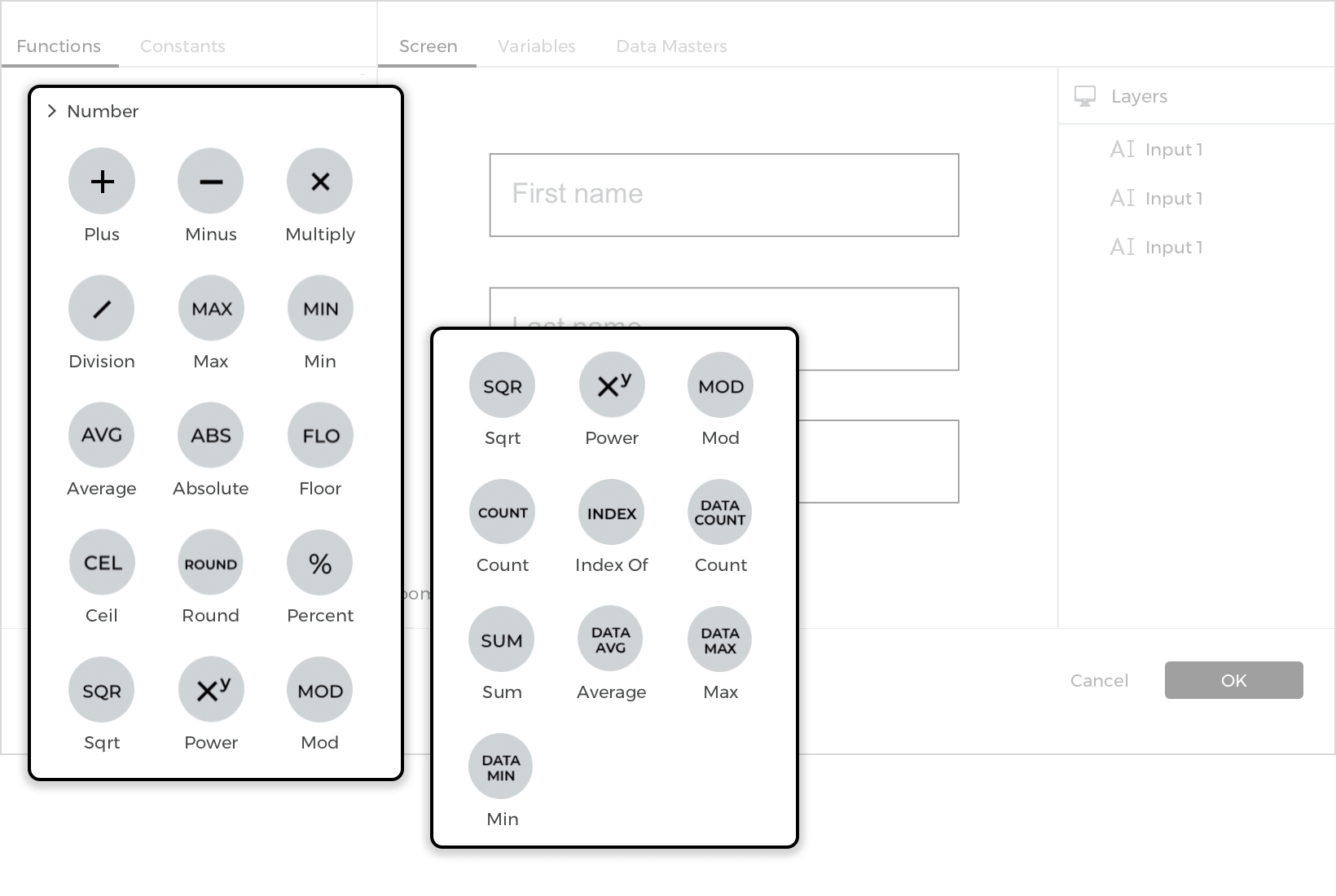• Plus: Takes two numbers and adds them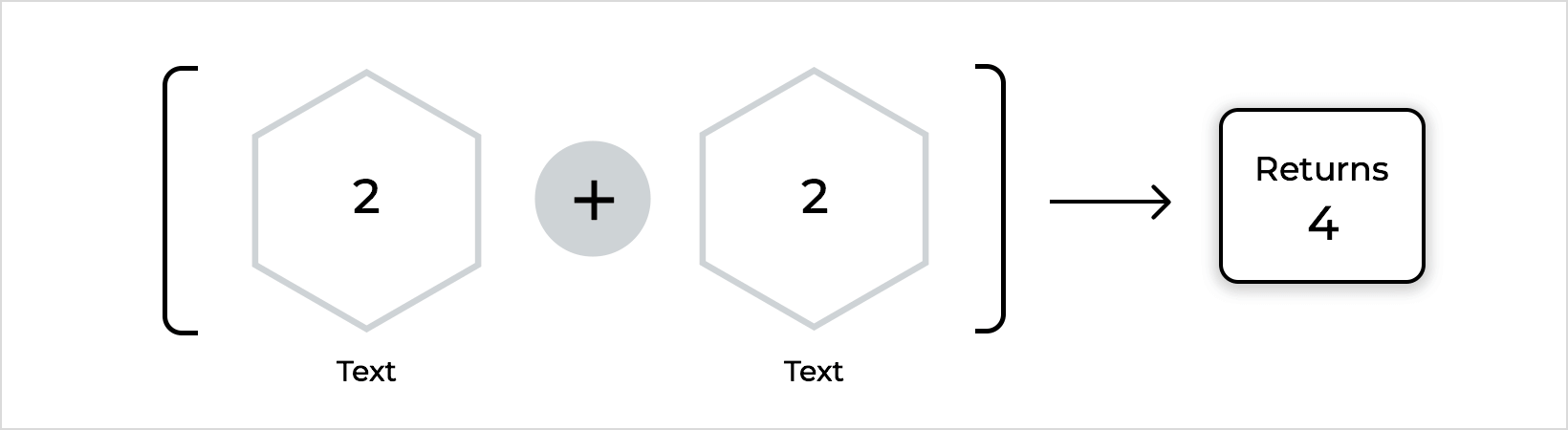• Minus: Takes two numbers subtracts the second from the first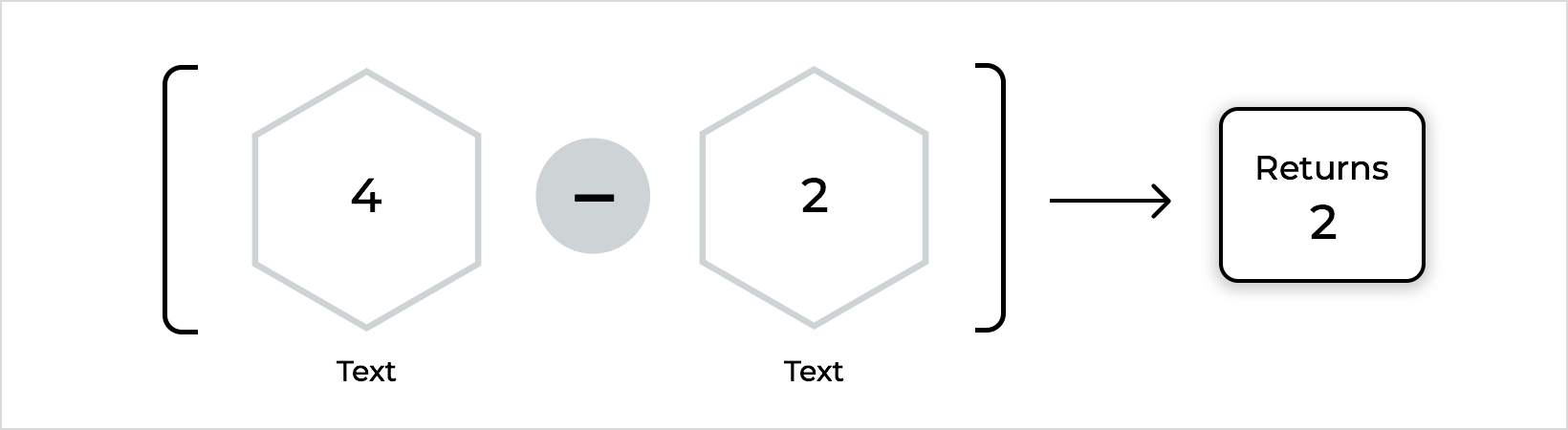• Multiply: Takes two numbers and multiplies them together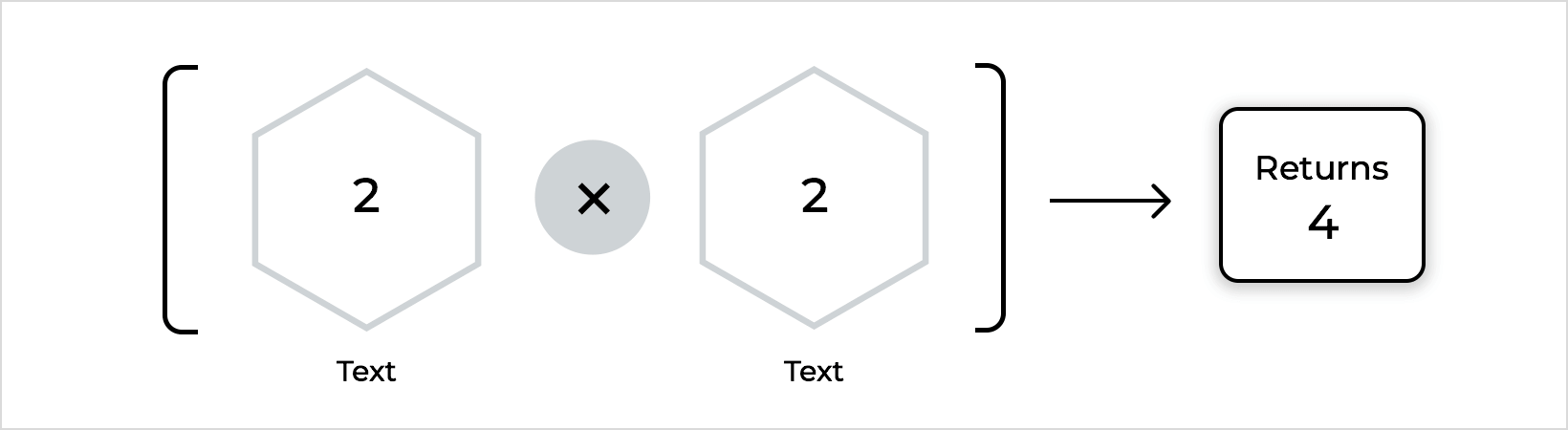• Division: Takes two numbers and divides the first by the second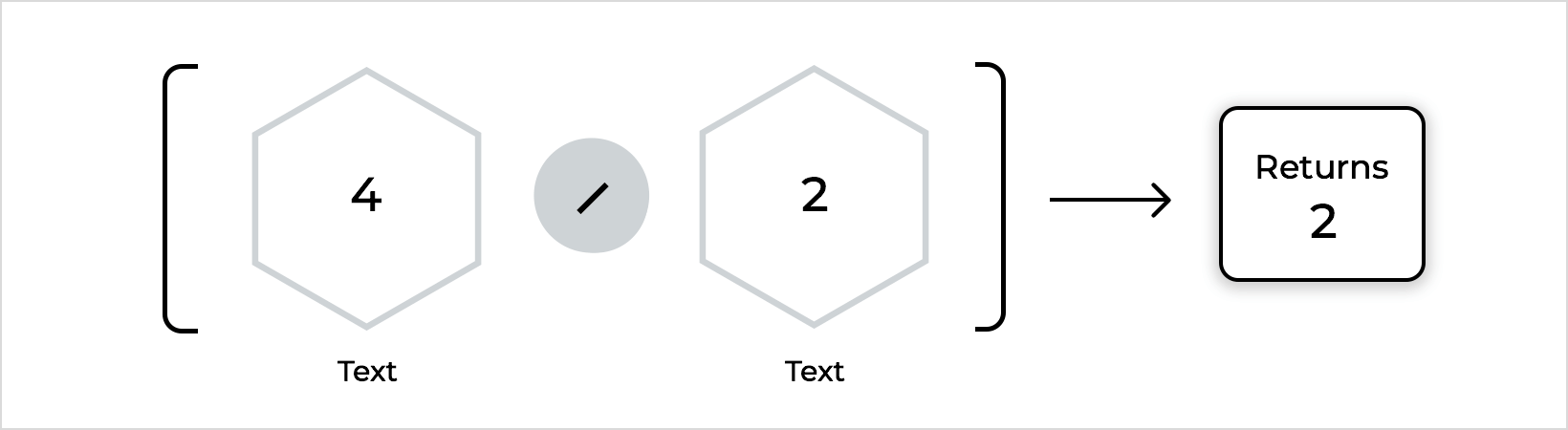• Max: Determines the greater of two numbers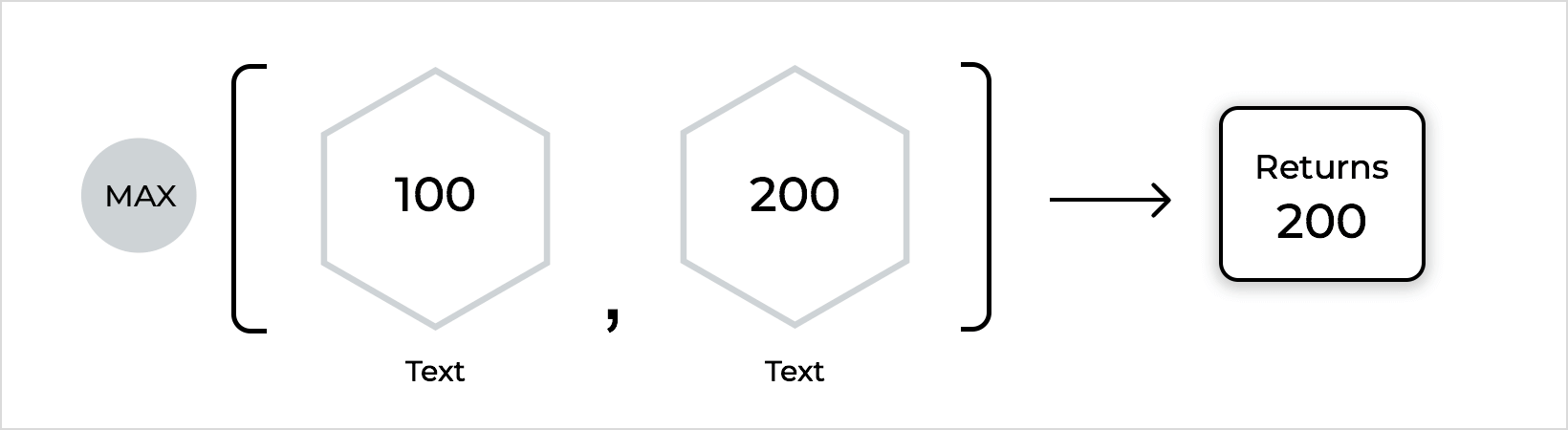• Min: Determines the smaller of two numbers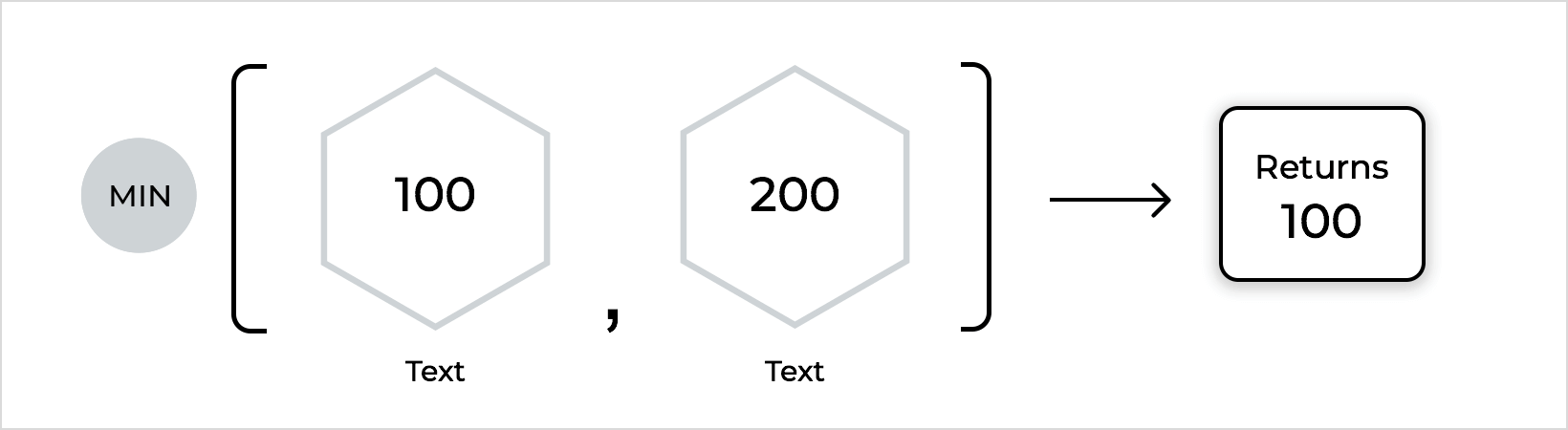• Average: Determines the average value of two numbers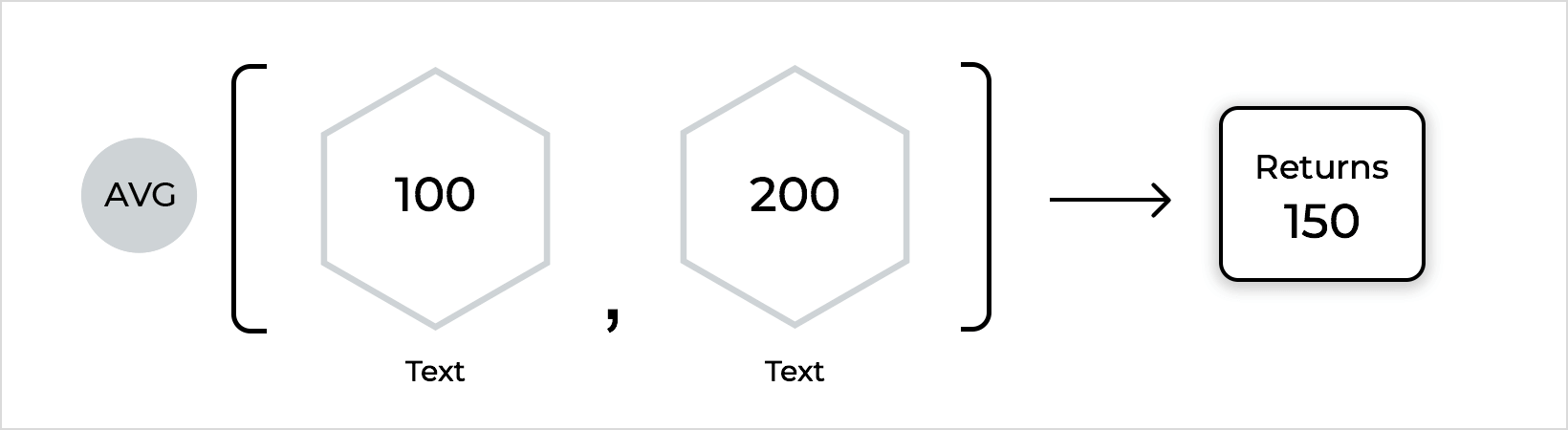• Absolute: Returns a number without its sign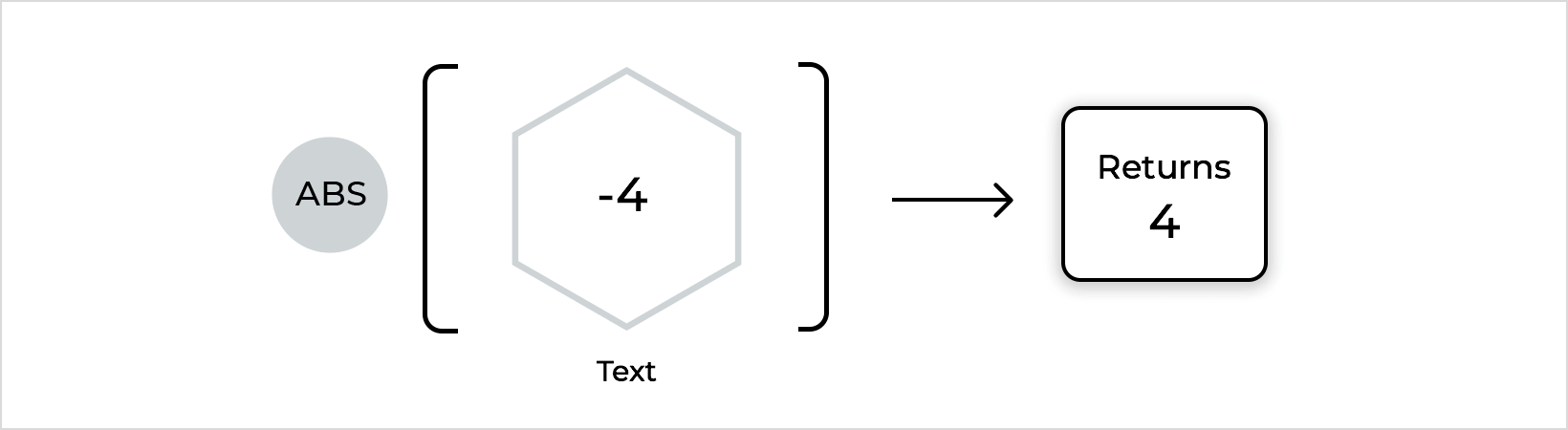• Round: Rounds a number to the number of decimals specified in the second space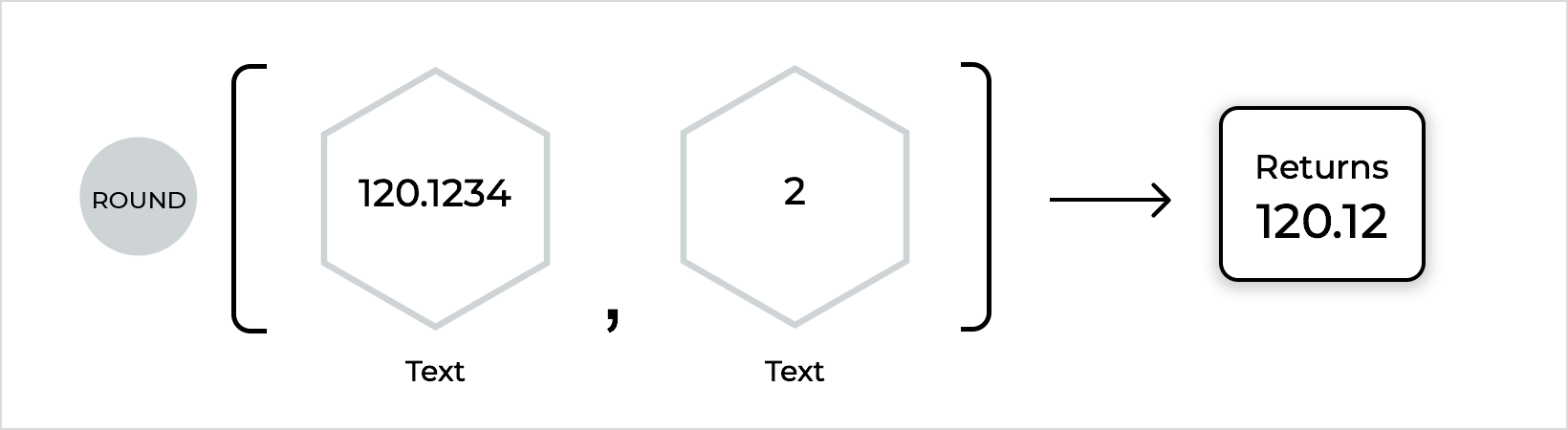• Percentage: Takes a percentage of the first number relative to the second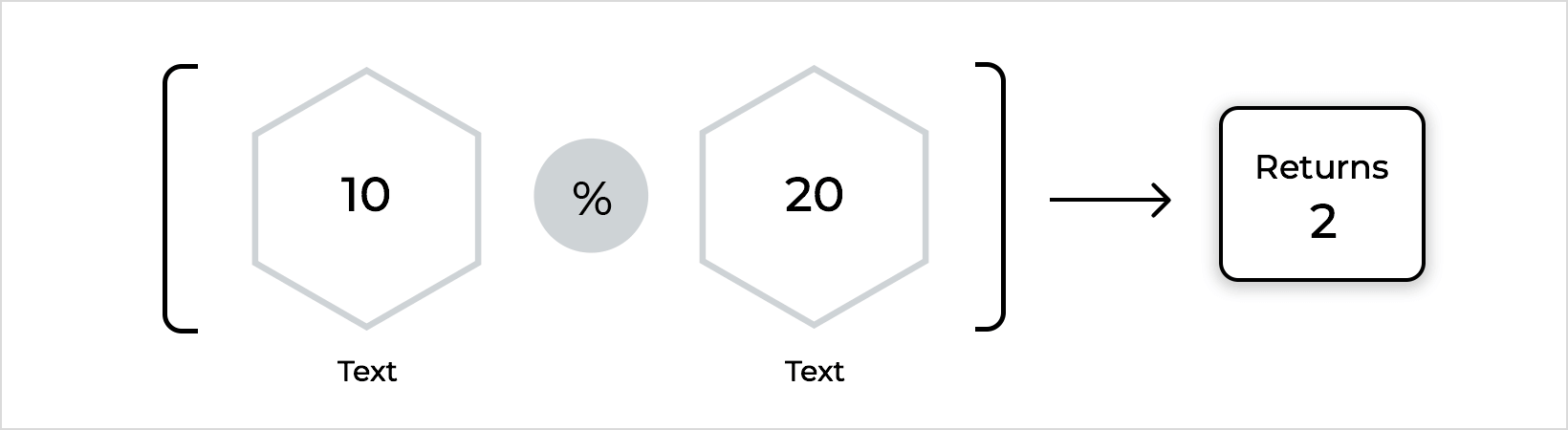• Sqrt: Gives the square root of the number entered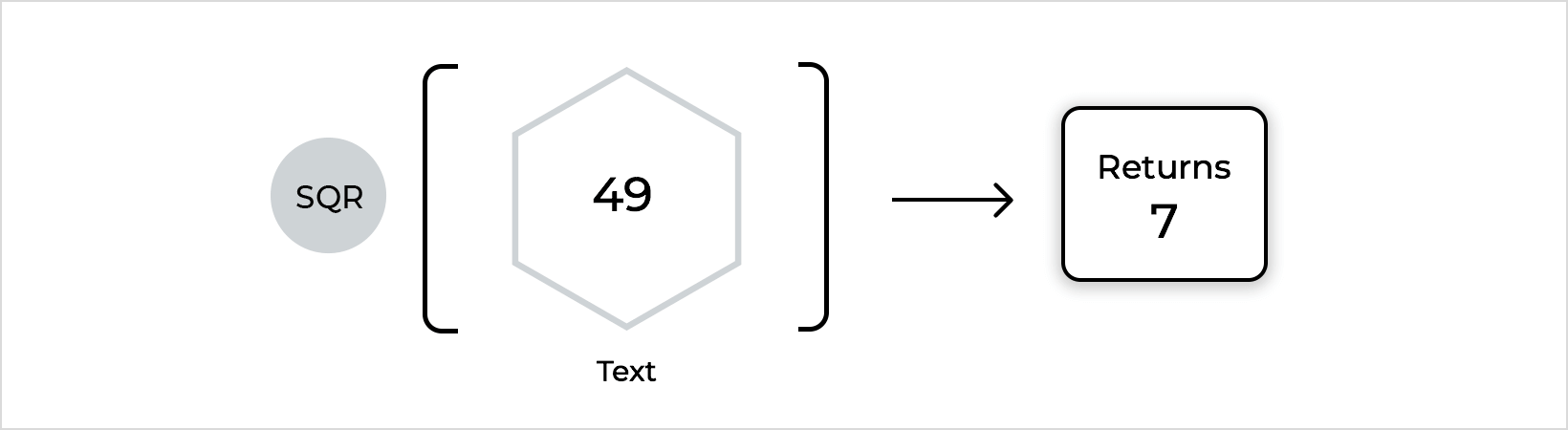• Mod: Returns the remainder after division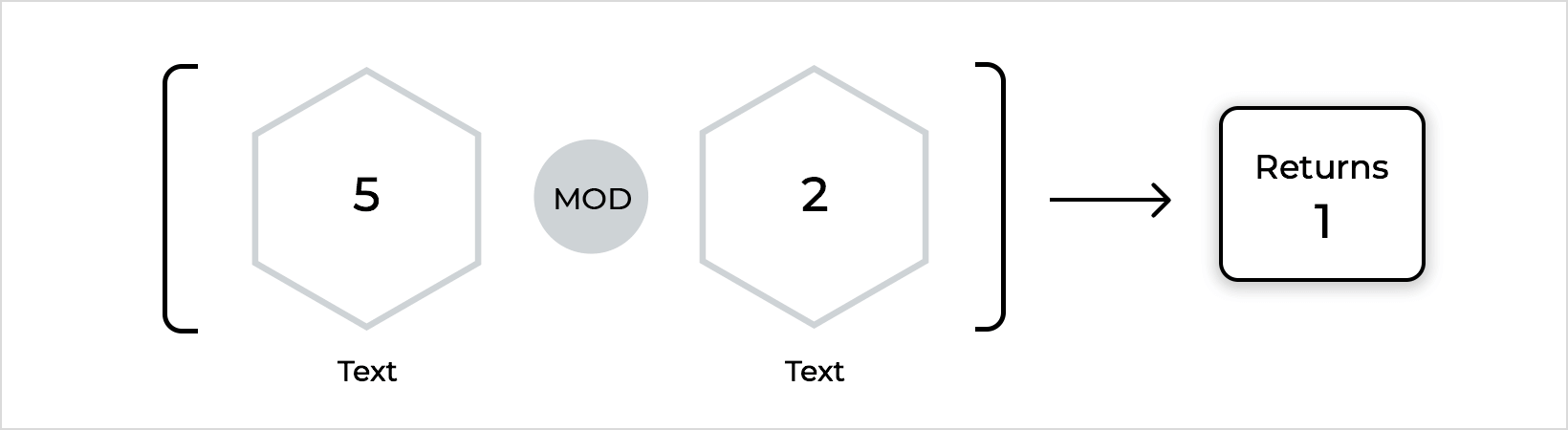### Comparators

Comparator functions relate to comparing two numbers or values: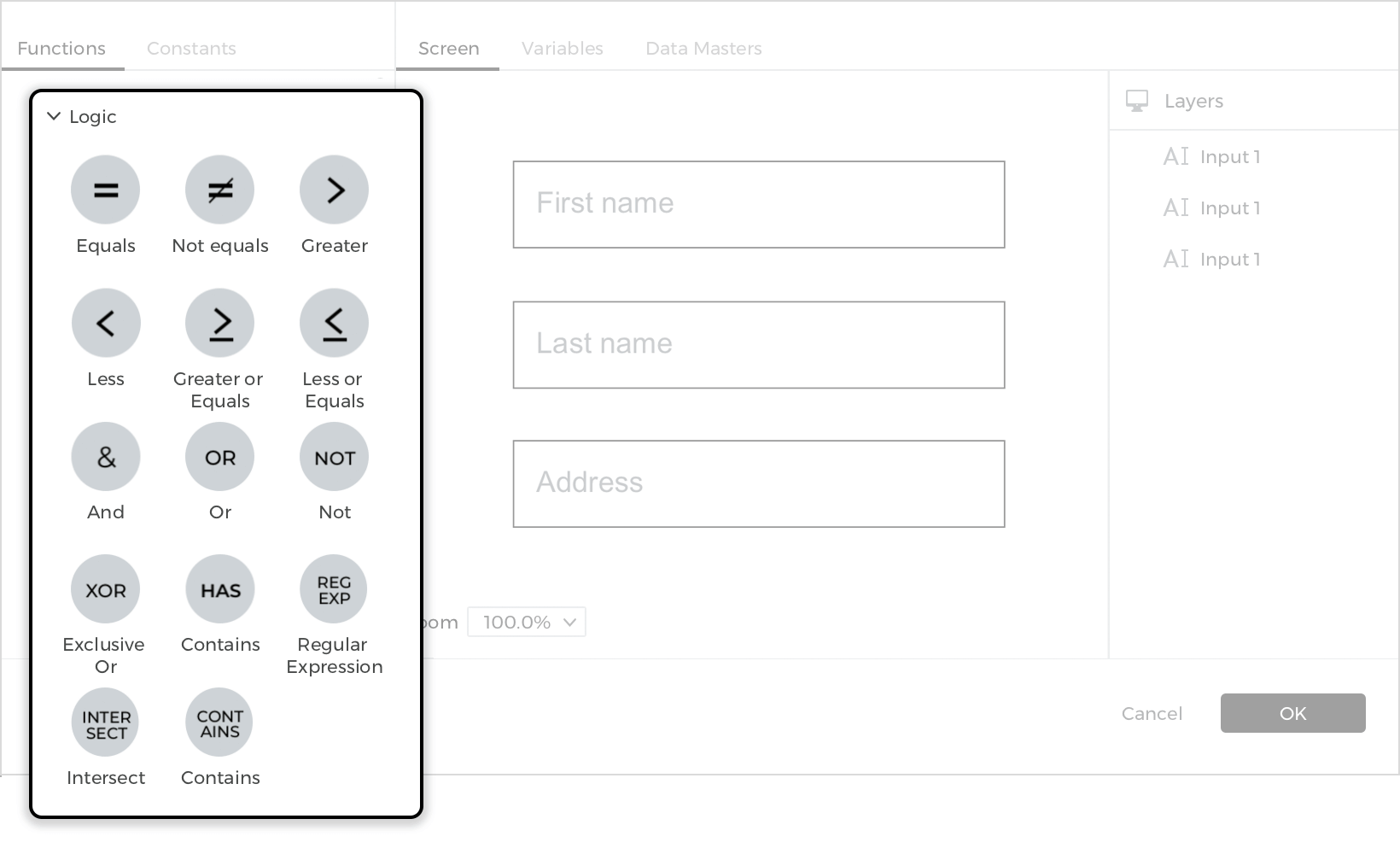• Equals: Returns true if both numbers have the same value
• Not equal: Returns true if the numbers don’t have the same value
• Greater: Returns true if the value of the number on the left is greater than the number on the right
• Less: Returns true if the value of the number on the left is smaller than the number on the right
• Greater or equal: Returns true if the value of the number on the left is greater than or equal to the number on the right
• Less or equal: Returns true if the value of the number on the left is smaller than or equal to the number on the right

### Text

Text functions will change or analyze characters in a text string: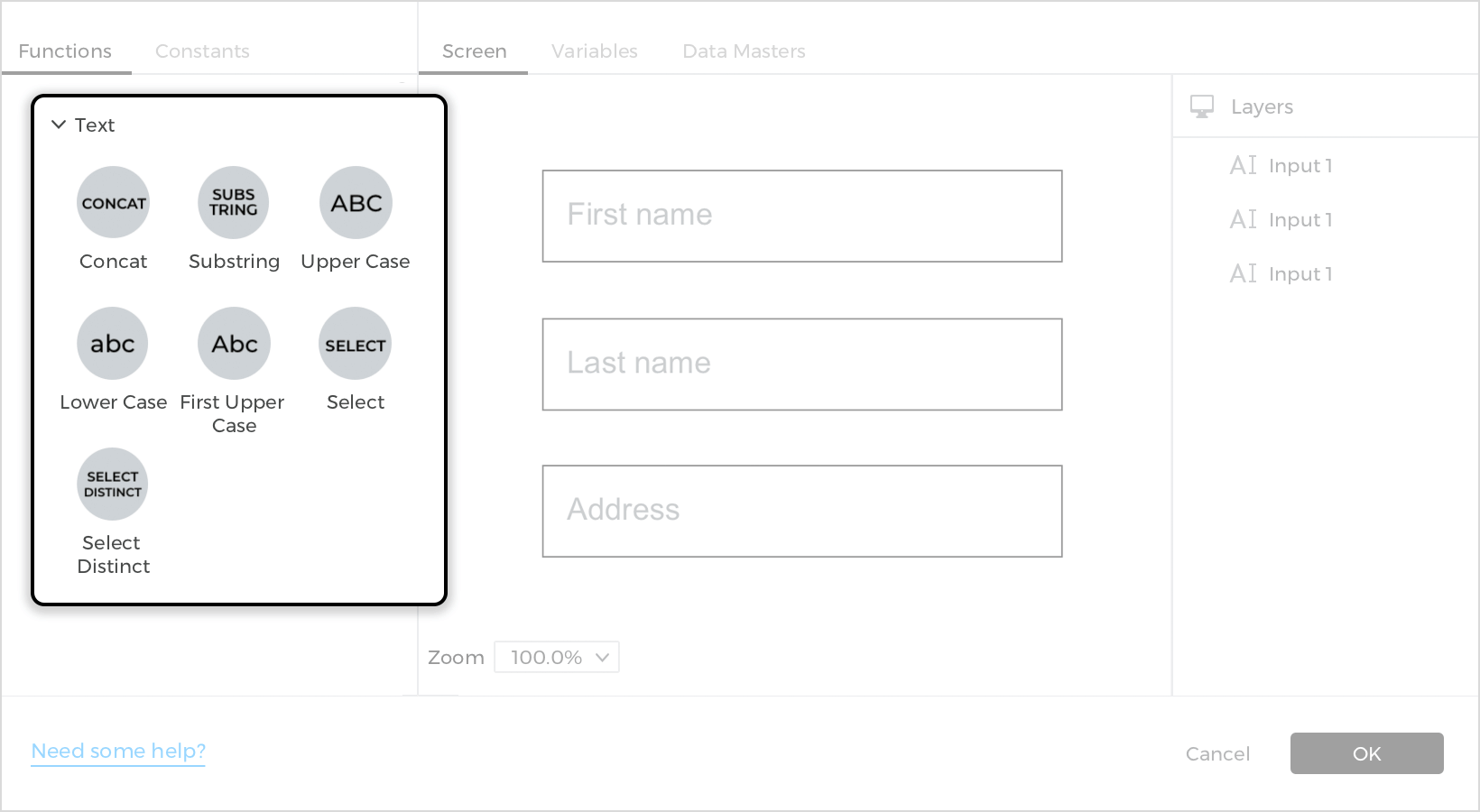• Count: Counts the number of characters in a value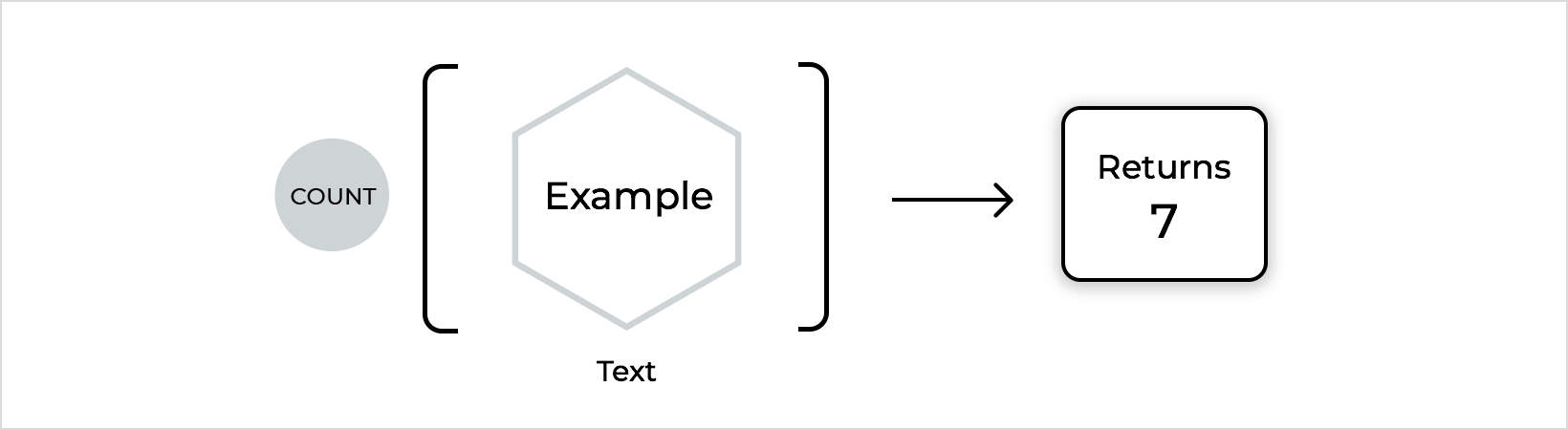• Concat (Concatenate): Combines two values, the first preceding the second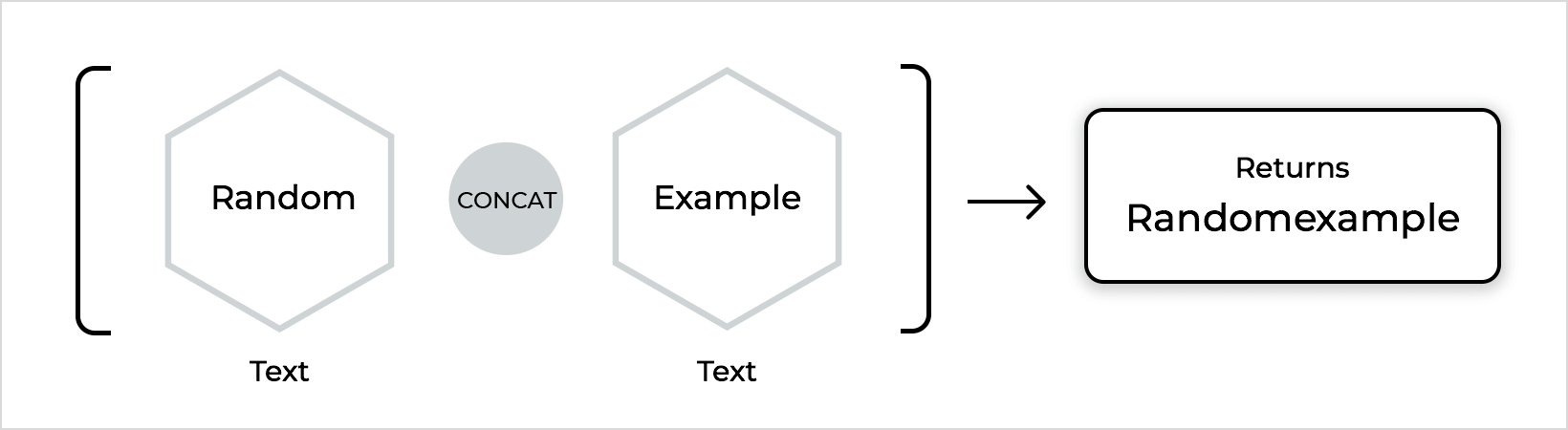• Substring: Extracts characters from text. The first space is the text, second is the starting position, third is the ending position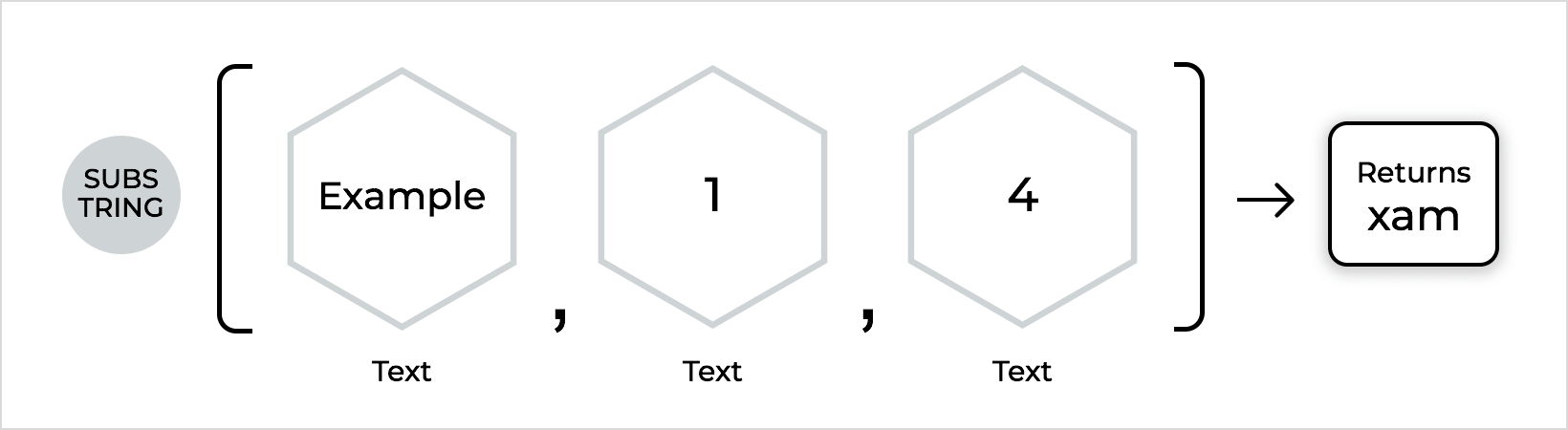• Index of: Returns a number that is the position of the second text within the first. If the second text is not found in the first, it will return -1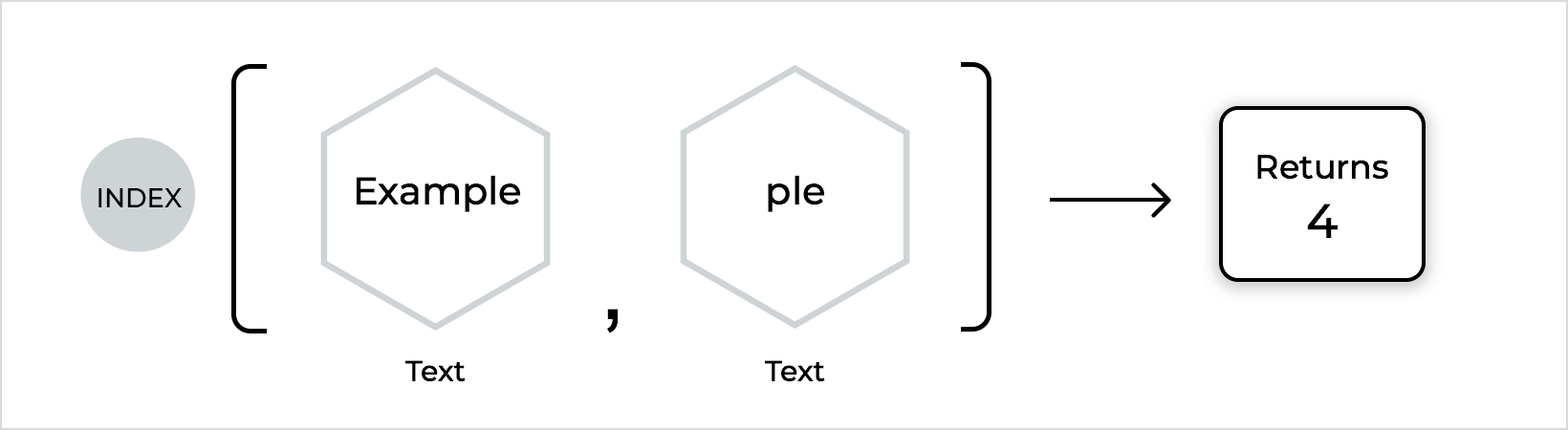• Upper case: Converts the characters to uppercase
• Lower case: Converts the characters to lowercase
• First upper: Capitalizes the first character
• Contains: Returns true if the first value contains the entire second value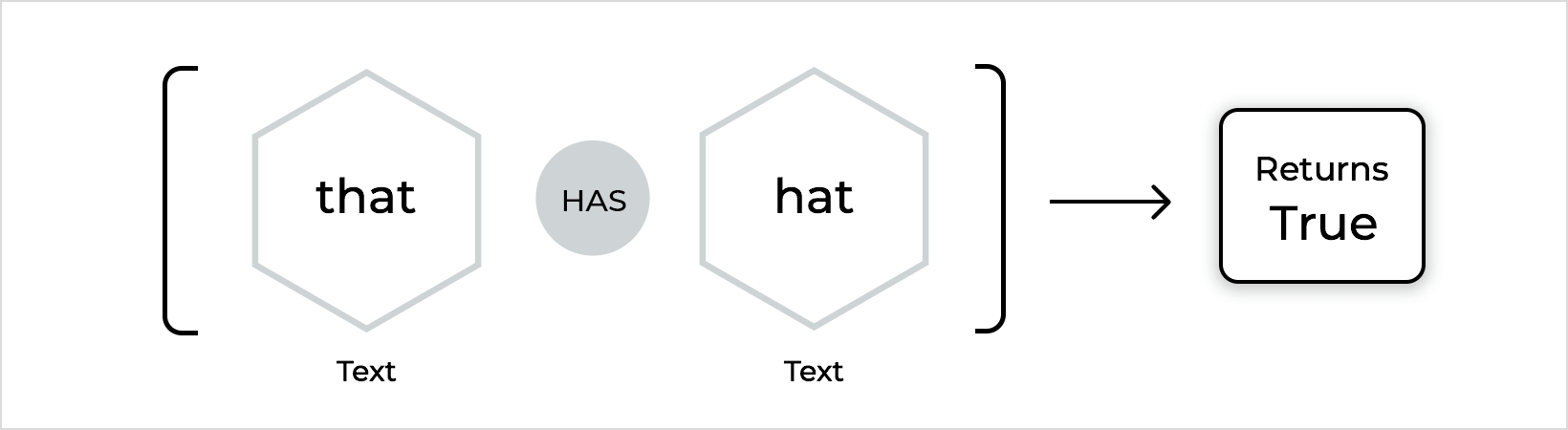• Regular expression: Checks if a value matches a regular expression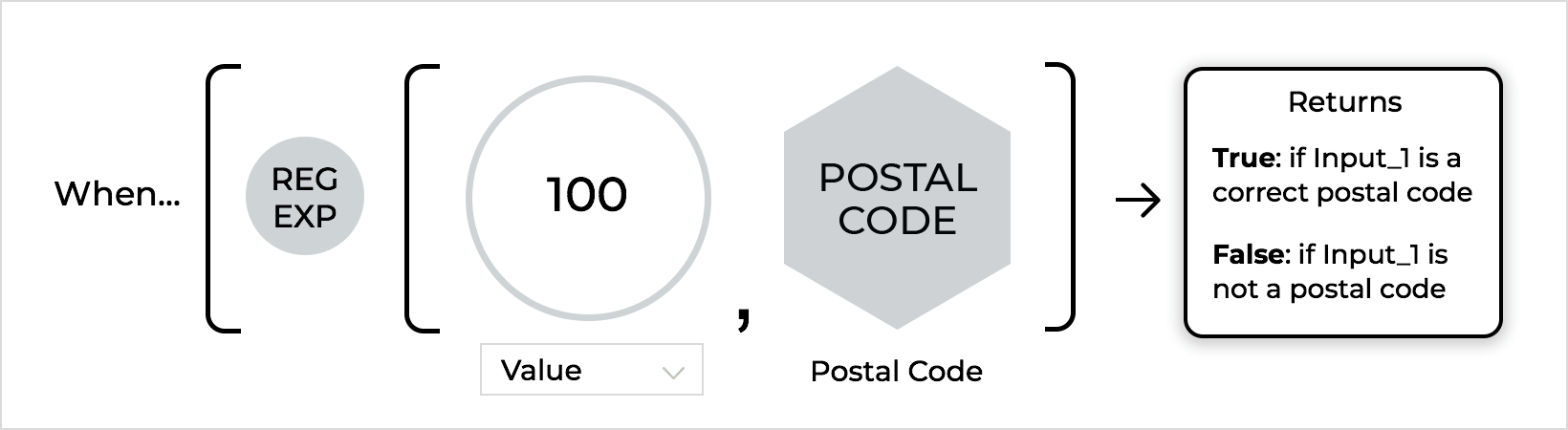### Logic

Logic functions relate to either/or arguments for strings.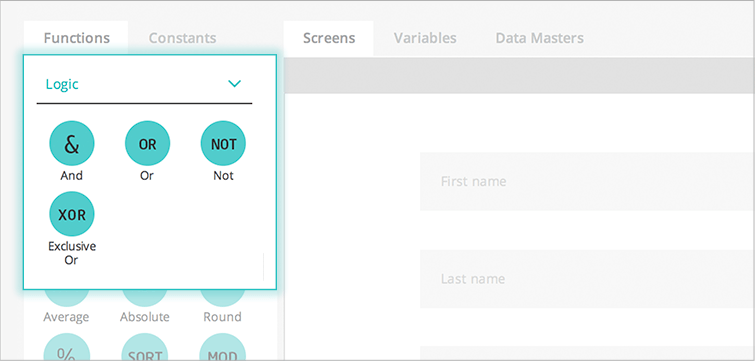• And: Returns “true” if all terms in a condition or value are true
• Or: Returns “true” if any term is true
• Not: Returns “true” if any term is not true
• Exclusive or: Returns “true” only if one of the terms is true

### Data

Data functions relate to data within Data Masters, Data Grids and Data Lists and other UI elements that contain data, such as Select Lists: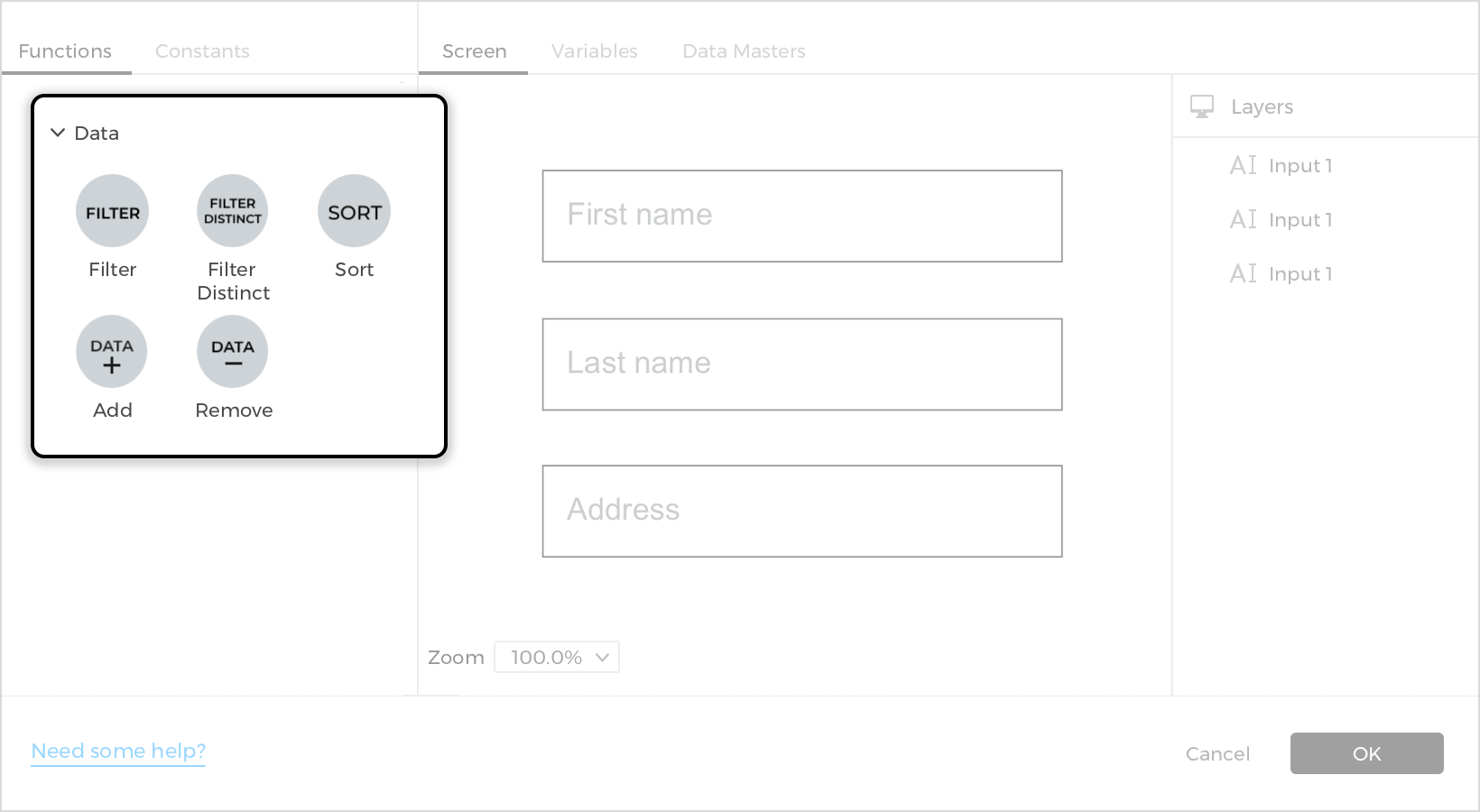• Select: Data function that selects a specific Data Master field from a row or record.
• The first space represents the row to select from (a record in a Data Master, Variable, or row of a Data List or Data Grid).
• The second space defines the Data Master field to select.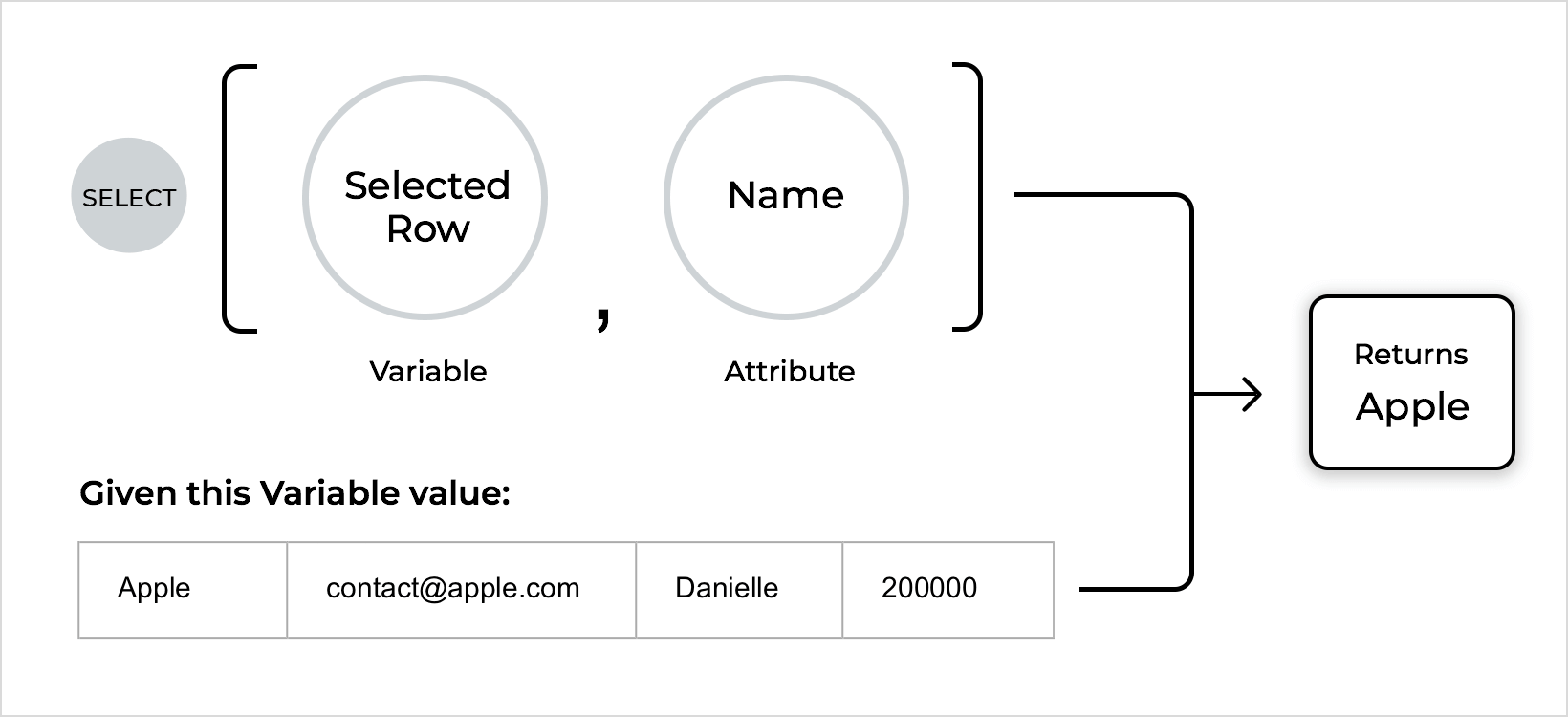• Filter: Data function that returns a list of rows or records according to a filter condition.
• The first space represents the data to select from (a Data Master, Variable, Data List, or Data Grid).
• The second space is the filter condition. Specify the records that should be included in the filter using logic functions or text.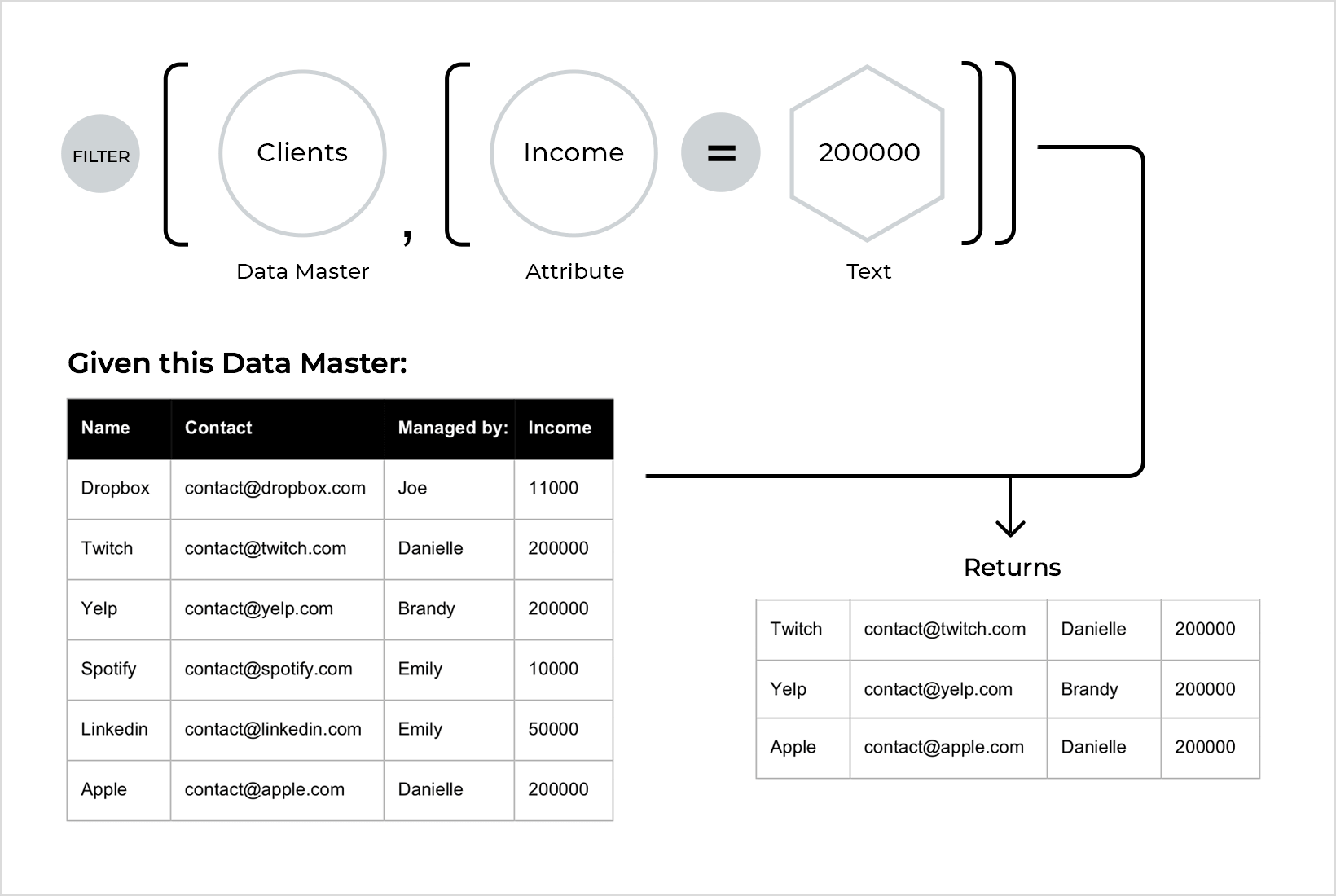• Select distinct: Finds and selects duplicate values from within a data set.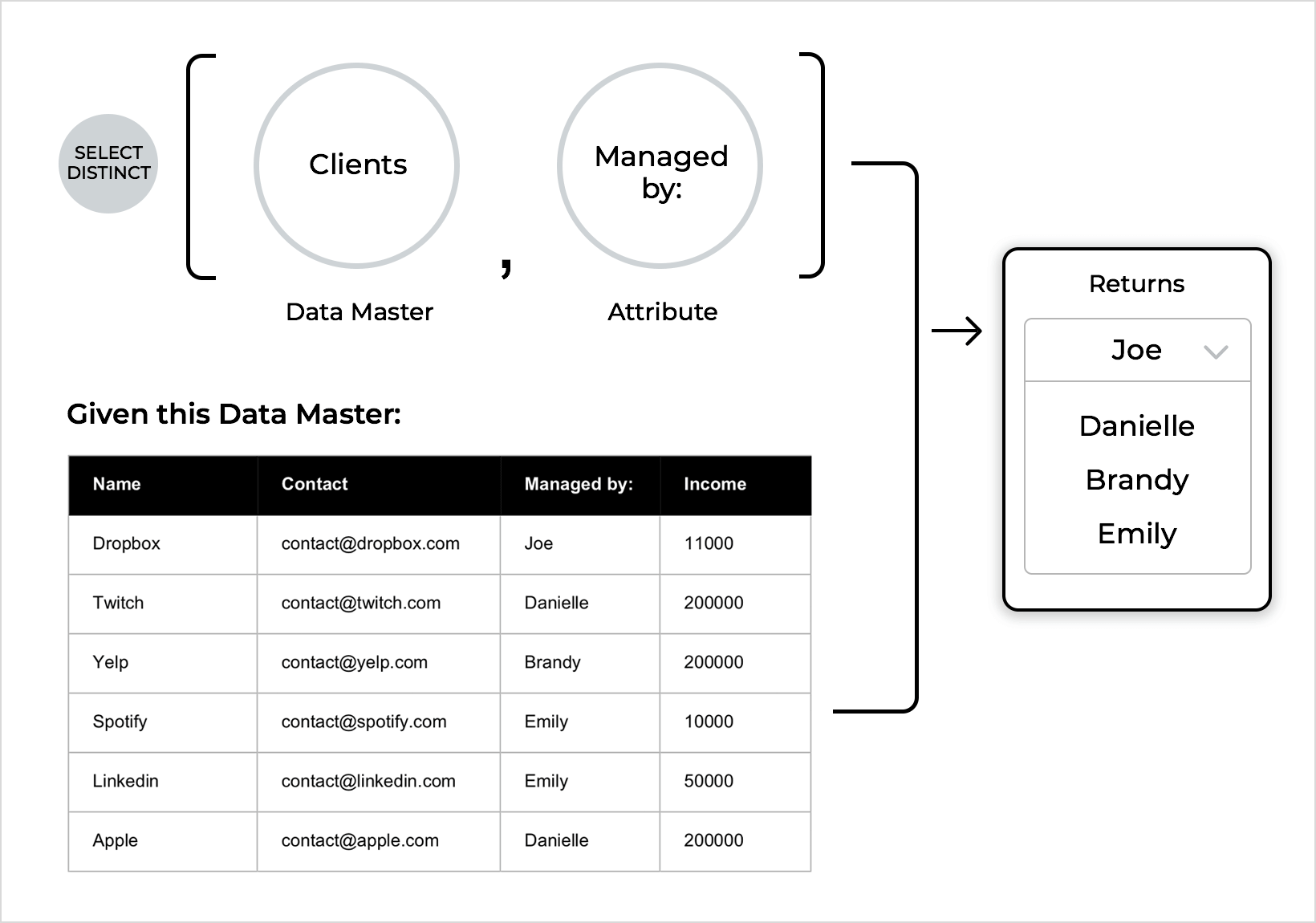• Filter distinct: Finds and filters duplicate values from within a data set.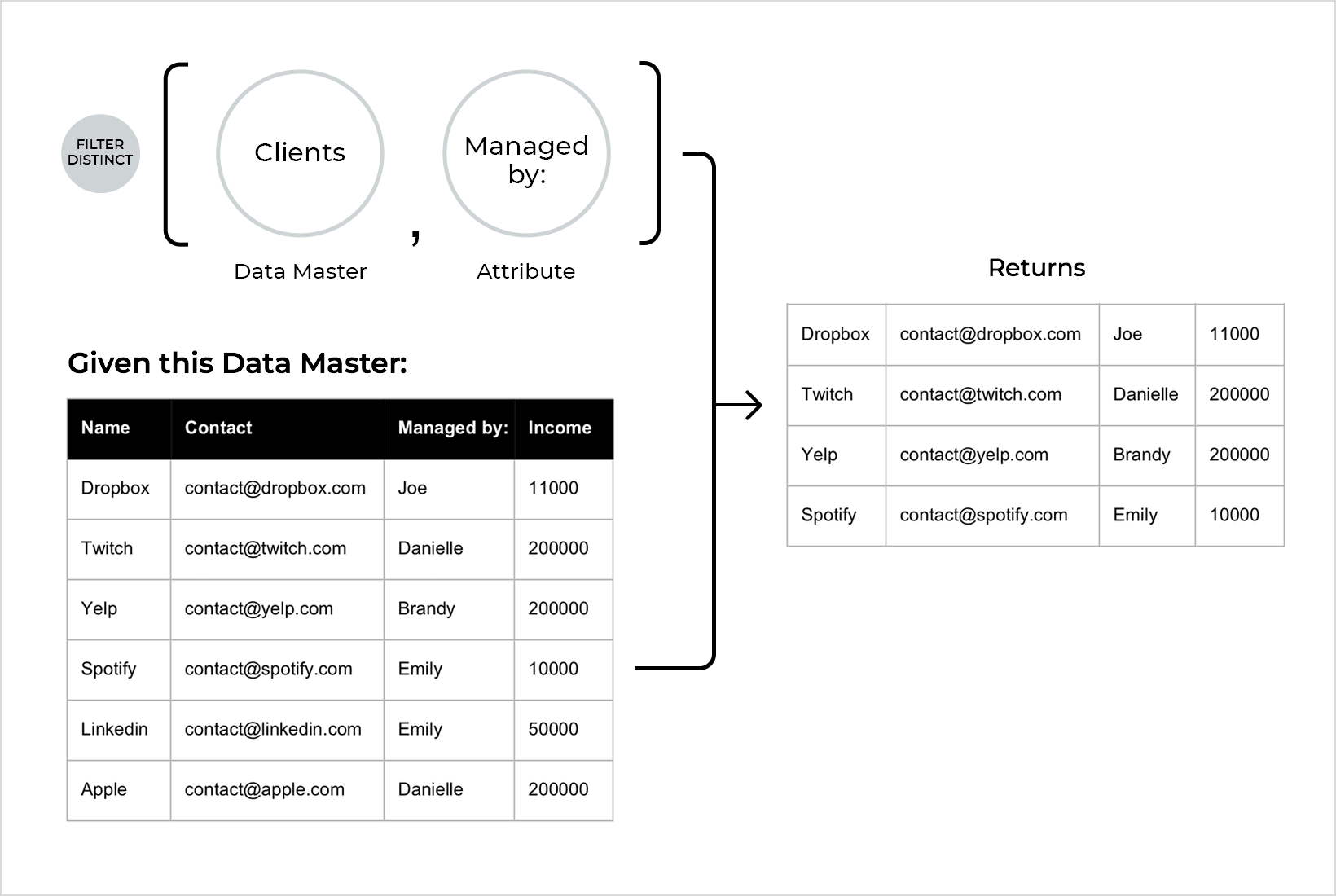• Count (data count): Returns the number of instances of a value included in a data set.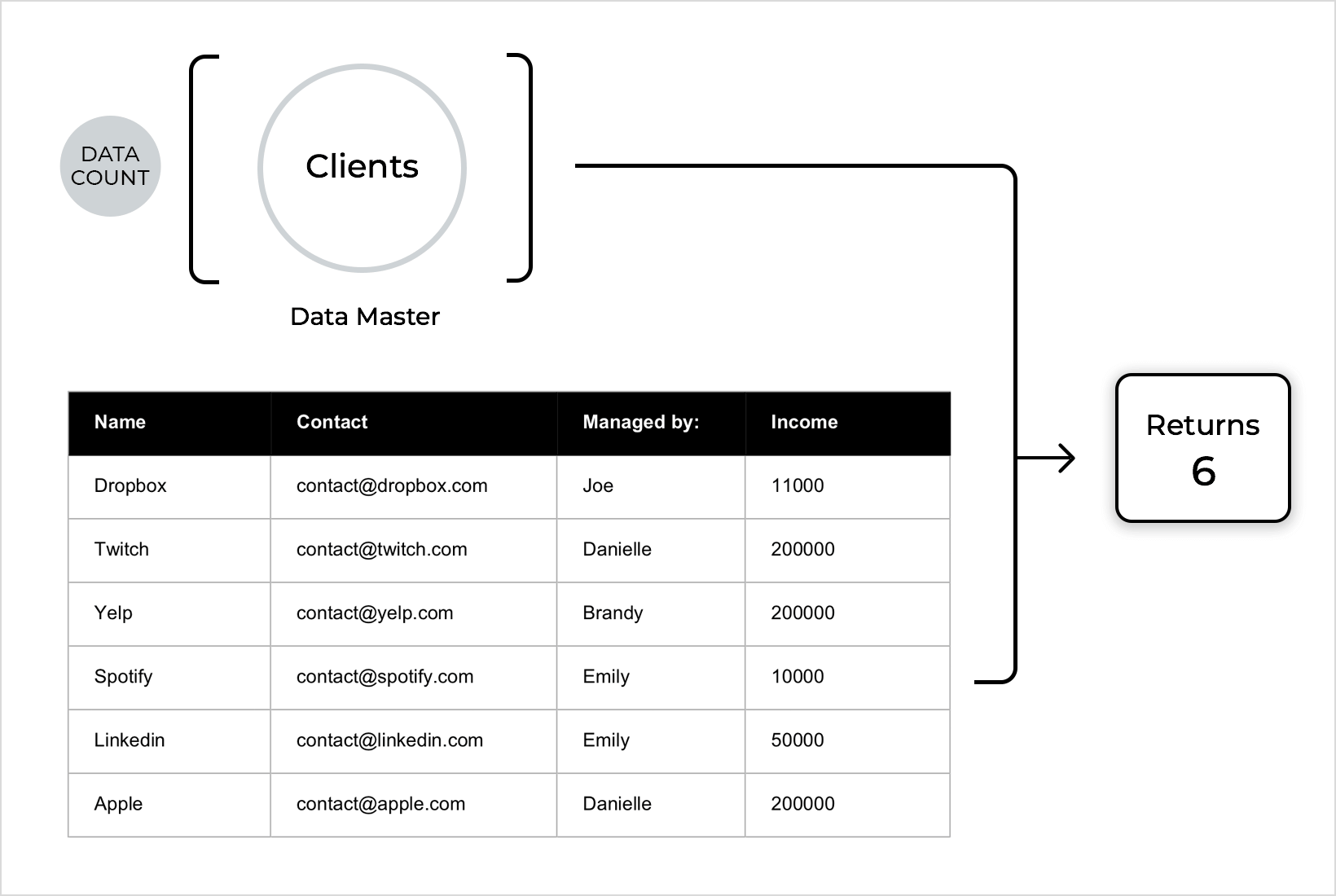• Sum: The sum of the values within a data set.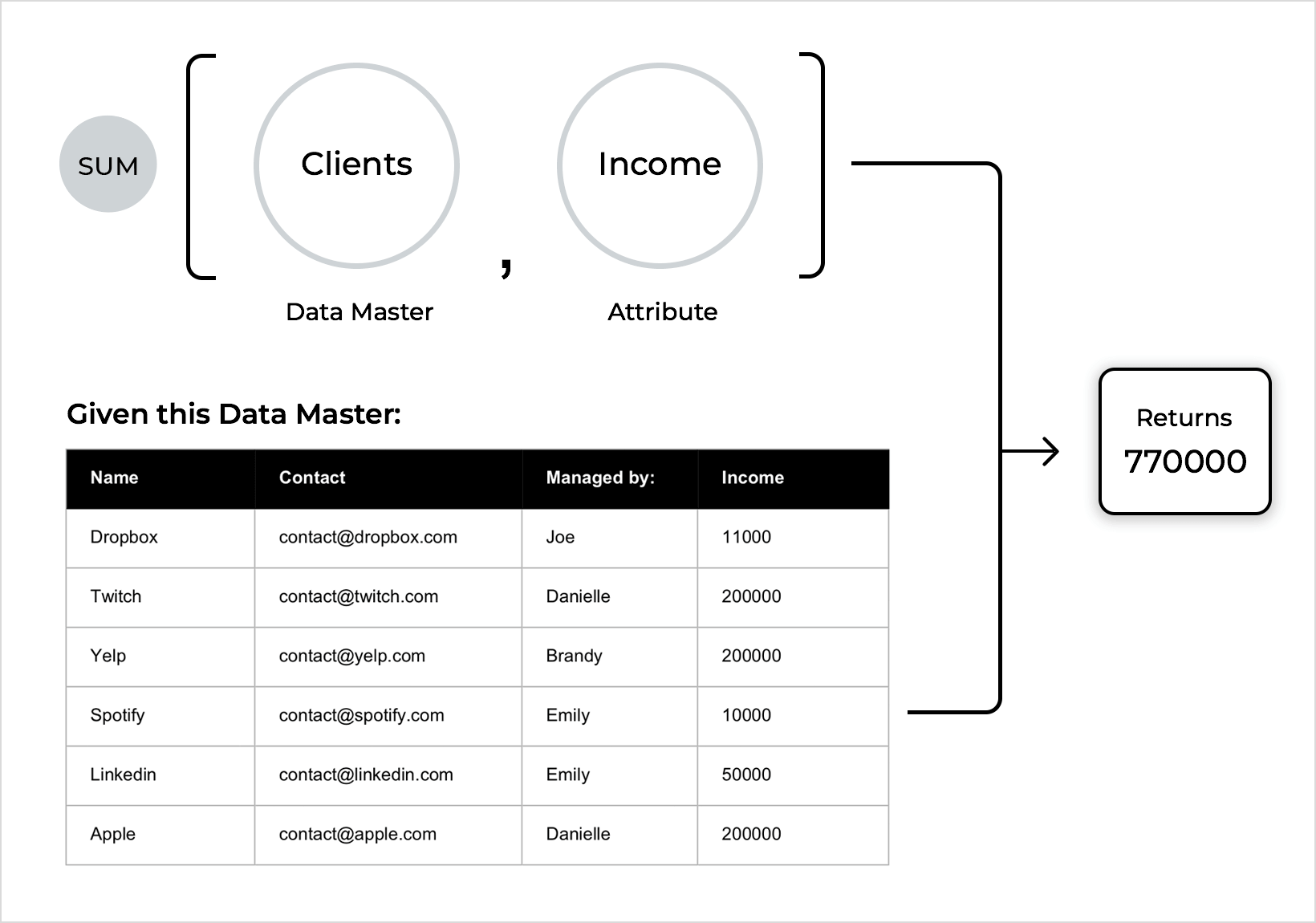• Average: Determines the average value of a data set
• Max: Determines the greatest number value from within a data set
• Min: Determines the smallest number value from within a data set
• Add (data plus): Combines two data sets together.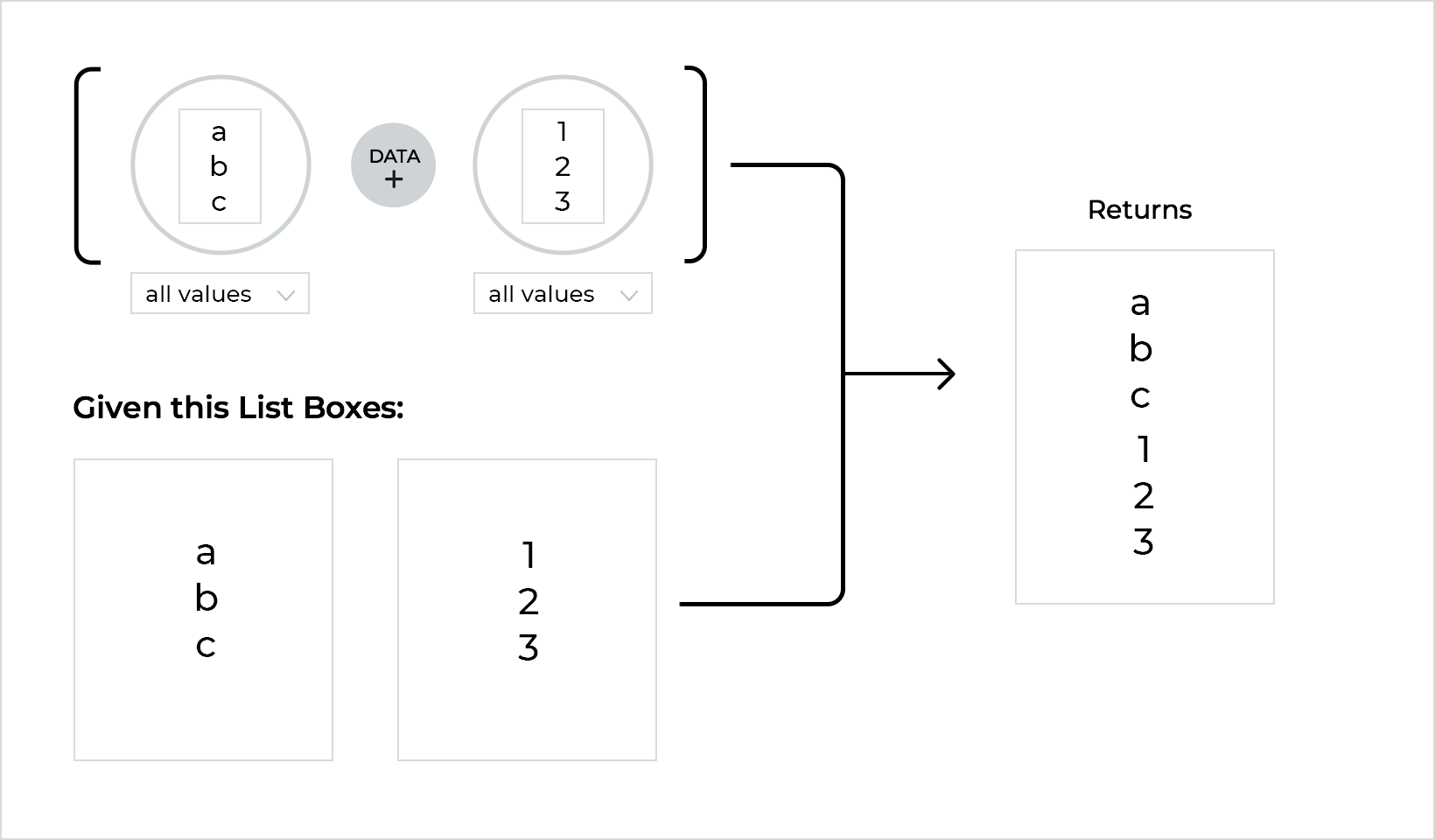• Remove (data minus): Removes the second data set from the first.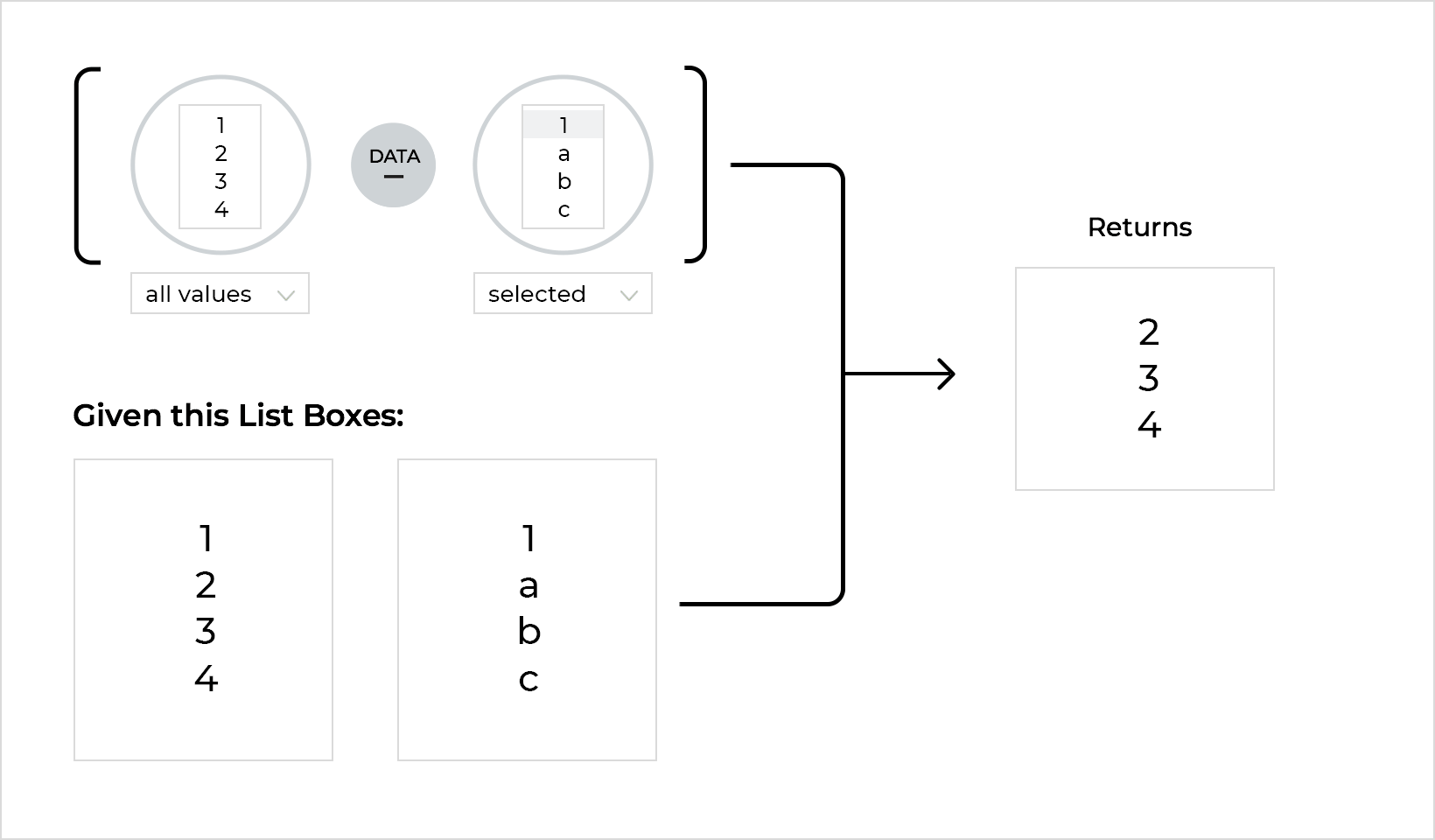• Sort: This function returns a list of sorted data. A drop-down appears to choose whether to list the data in ascending or descending order. Two spaces of data are shown in the expression.
• The first represents a set of data to be sorted (e.g. a Data List row).
• The second represents the data that the first will be sorted by (e.g. a Data Master field)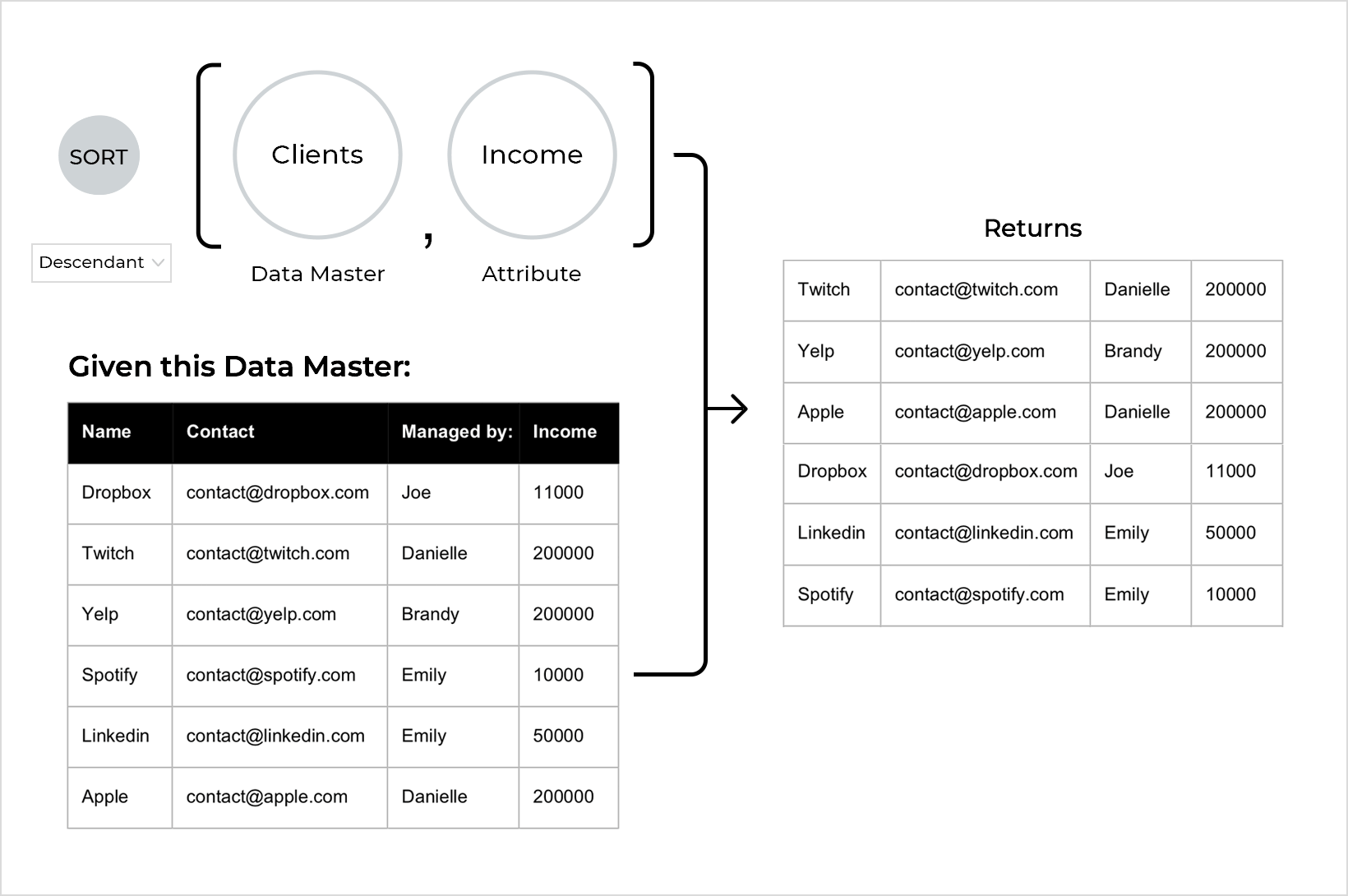### Areas

Area functions determine if two elements are touching, or if one contains another: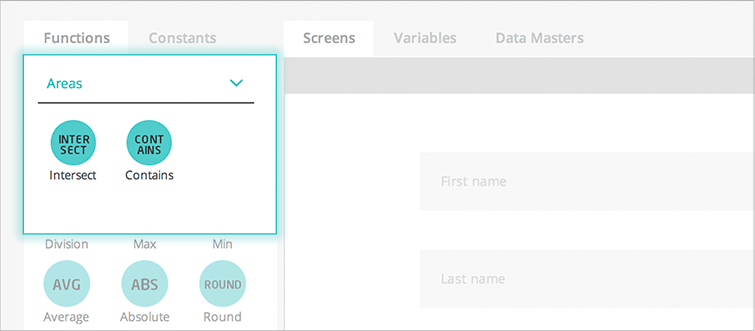• Intersect: Determines if two elements are touching
• Contains: Determines if an element contains another

## Constants in the expression builder

Constants are divided into two categories: Constants and Regular Expressions.

### Constants

Standalone constants, such as a text, the date and time, screen properties and mouse cursor position can be used to define conditions and create values.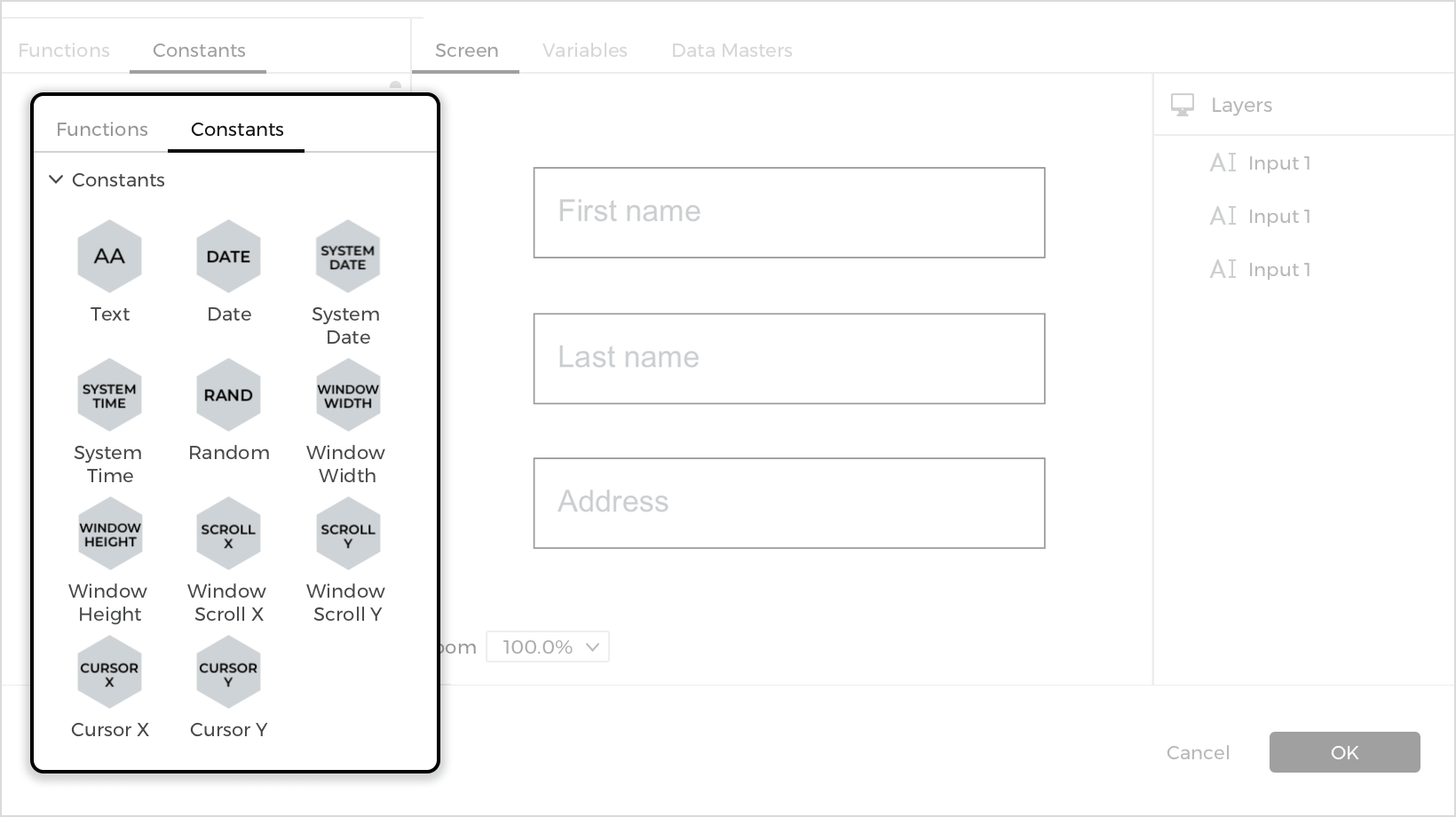• Text: Input any (and an unlimited number of) characters
• Date: Displays a date selector
• System date: Displays the system date
• System time: Displays the system time
• Random: Generates a random number between 0 and 1
• Window width: Displays the width of the Canvas in pixels
• Window height: Displays the height of the Canvas in pixels
• Window scroll X: Displays the Canvas’ horizontal scroll position
• Window scroll Y: Displays the Canvas’ vertical scroll position
• Cursor X: Displays the mouse cursor’s vertical scroll position
• Cursor Y: Displays the mouse cursor’s horizontal scroll position

### Regular Expressions

Regular expressions are used to ensure that values meet ‘rules’ (e.g. to ensure that a zip code is correct in a registration form).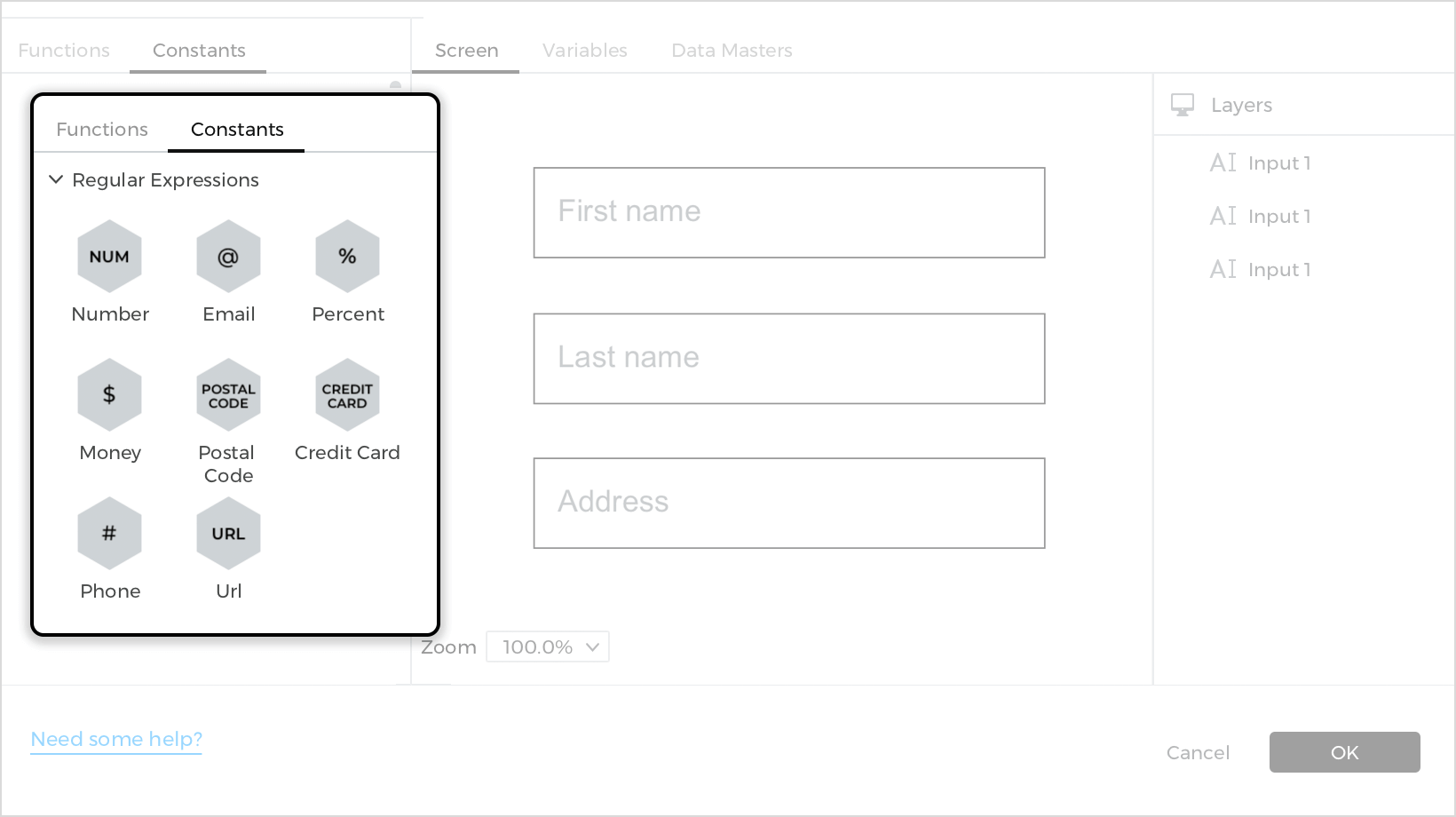• Number: Displays the number string entered by the user, with or without decimal points (separated by ‘.’ or ‘.’)
• Email: Displays the email address entered by the user (must contain a username, “@”, a valid server and “.com”)
• Percentage: Displays the percentage entered by the user, with or without decimal points of up to two decimal places
• Money: Displays the monetary value entered by the user. Decimal points and currency signs are optional
• Postal code: Displays the US five-digit zip code entered by the user
• Credit card: Displays any major credit card
• Phone: Displays any number between six and 10 digits (with or without spaces and/or dashes)
• URL: Displays a URL

## UI element options in the expression builder

Drag and drop UI elements from the current screen to the expression to build a condition or value: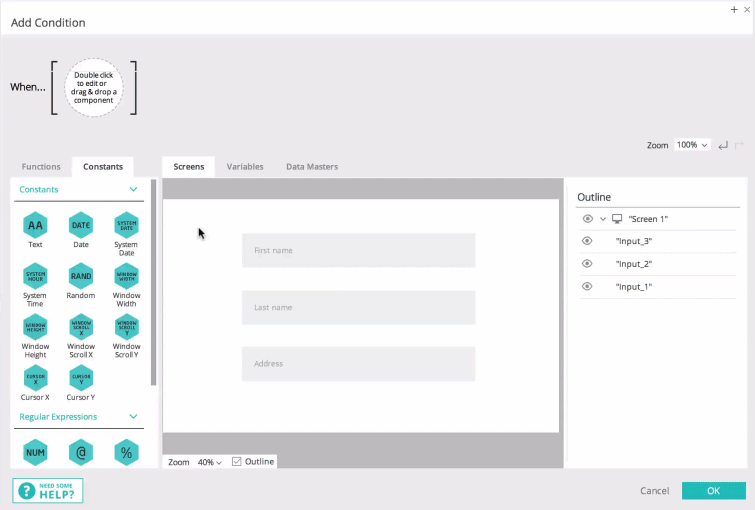Then, choose which attribute of the element is to be used in the expression. Choose from the following options:

• Value: The current selected value of an element on the current screen. Almost all widgets will include this option
• Pos.X relative: The X coordinate of the selected element’s position relative to its closest parent container
• Pos.X absolute: The X coordinate of the selected element’s position relative to the screen
• Pos.Y relative: The Y coordinate of the selected element’s position relative to its closest parent container
• Pos.Y absolute: The Y coordinate of the selected element’s position relative to the screen
• Width: The width of the element in pixels
• Height: The height of the element in pixels
• Angle: The rotation angle of the element in degrees
• Is visible: Visible only if the element is visible
• Area: The measurements of an element (see Area functions above)
Note: both the X and Y positions, absolute or not, refer to the top-most and left-most positions of the element respectively

## Variables in the expression builder

You can also drag and drop variables to the expression, and use them to create advanced conditions or values that can be shared between different screens in a prototype.